# Data Visualization

This chapter will cover plotting functions from the ggplot2 package.

## Create a Plot

We first create an initial ggplot object using ggplot() function. We declare the data for plotting within ggplot(), then add layers/components (such as geom functions) to the plot.

To construct a scatterplot, for instance, we add geom_point() with an aesthetic mapping argument inside the parentheses. The aesthetic mappings aes() describe how variables in the data are mapped to visual properties of geometric objects (geom).

``````## ggplot(data = data_object, mapping = aes(global aesthetics)) +
##   <geom_function>(mapping = aes(specific aesthetics))

ggplot(data = hsb) +   # create a ggplot
geom_point(mapping = aes(ses, mathach))  # add a layer of points (scatterplot)``````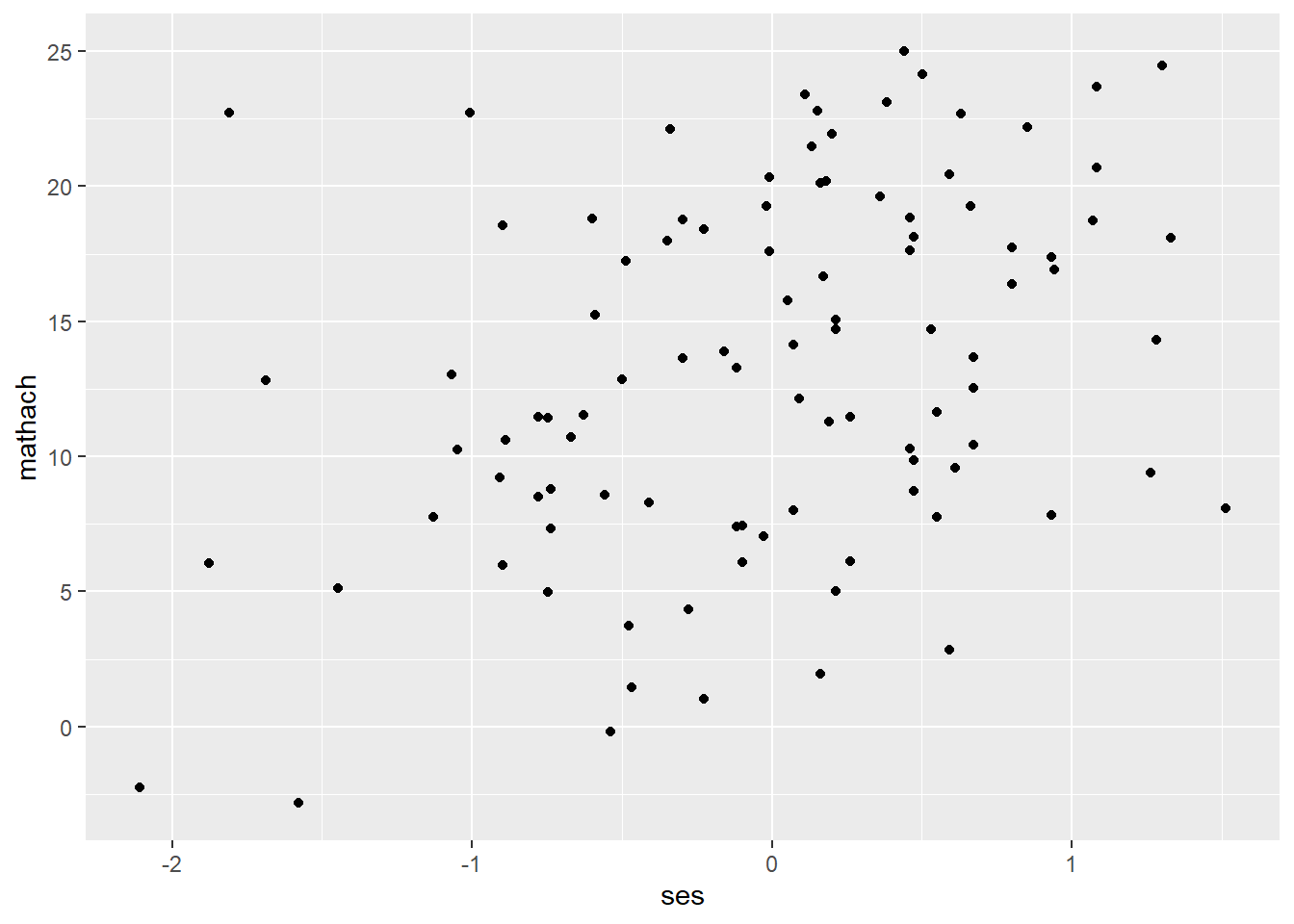``````# or

ggplot(data = hsb, mapping = aes(ses, mathach)) +
geom_point()   ````````````## You can also use the pipe operator

hsb %>%    # data
ggplot() +     # create a ggplot
geom_point(mapping = aes(ses, mathach)) # add geom_function()``````## Geom Functions

Frequently used geom functions are introduced in this section. More functions can be found here: https://github.com/rstudio/cheatsheets/blob/master/data-visualization-2.1.pdf

< One variable >

• geom_histogram() for a continuous variable
``````ggplot(data = hsb) +
geom_histogram(mapping = aes(mathach), bins = 25)
#or ``````
``````ggplot(data = hsb, mapping = aes(mathach)) +
geom_histogram(bins = 25) # can set the number of bins``````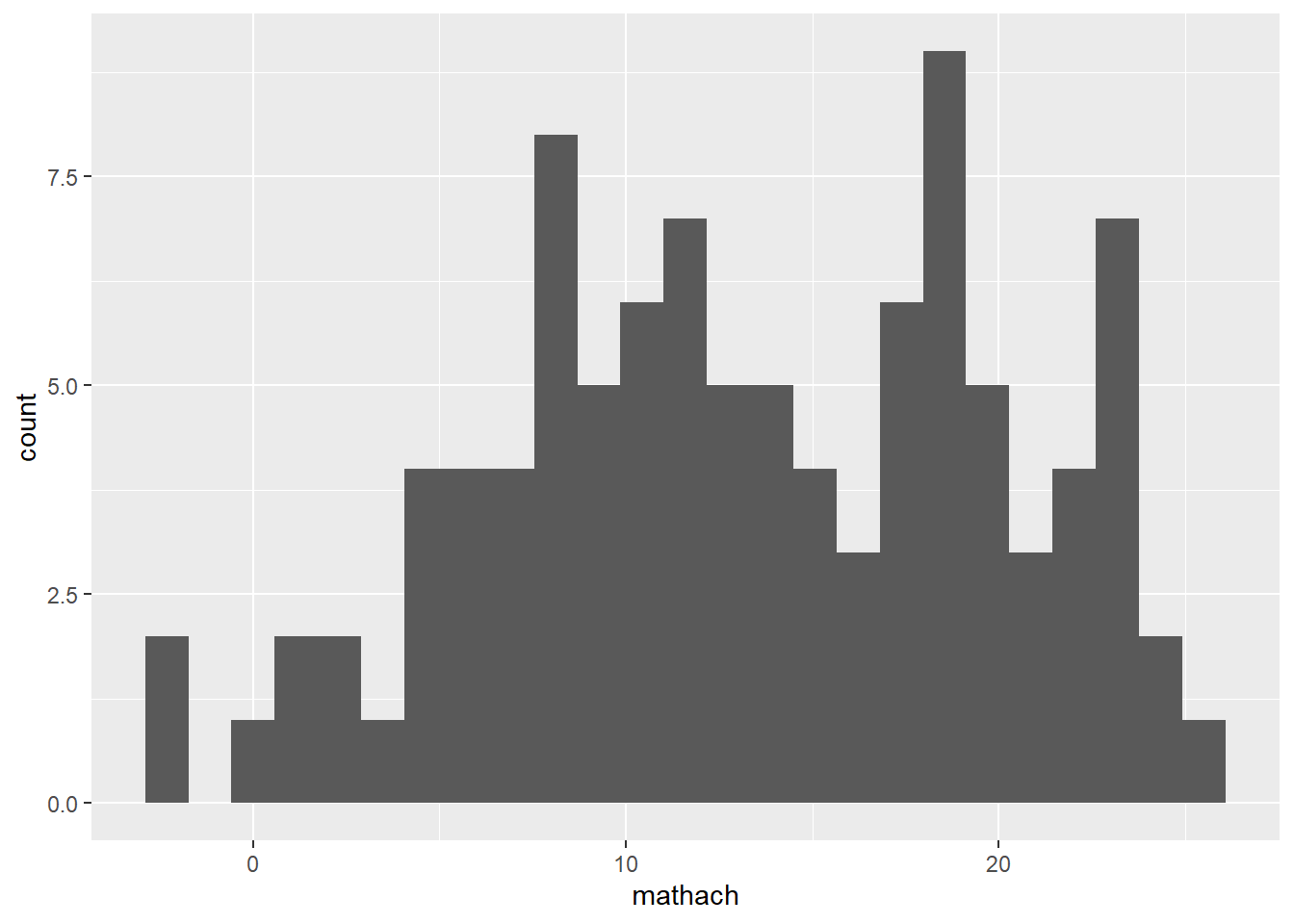• geom_density() for a continuous variable
``````ggplot(data = hsb) +
geom_histogram(mapping = aes(mathach))
# or ``````
``````ggplot(data = hsb, mapping = aes(mathach)) +
geom_density() ``````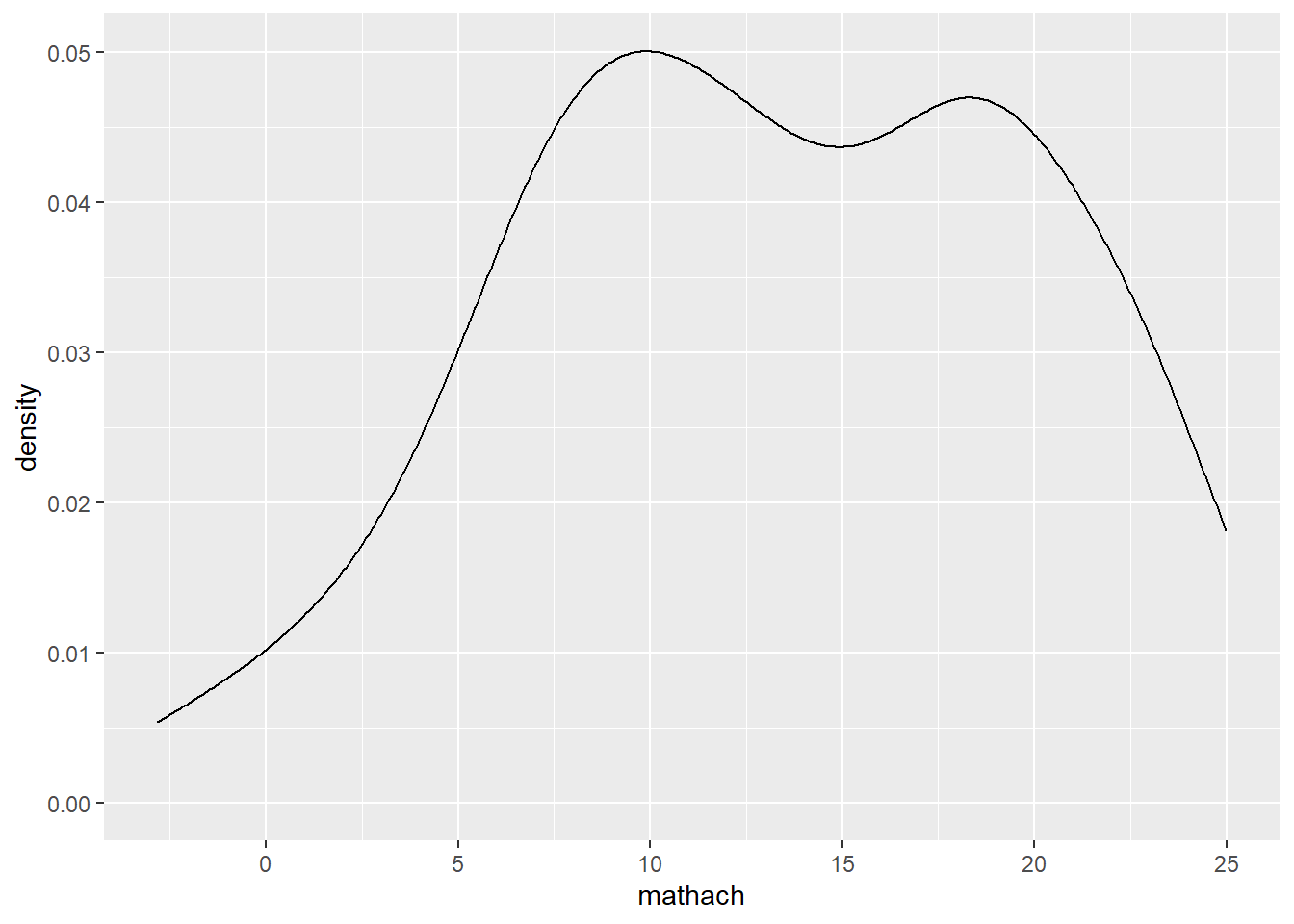• geom_bar() for a discrete variable
``````ggplot(data = hsb, mapping = aes(female)) +
geom_bar() ``````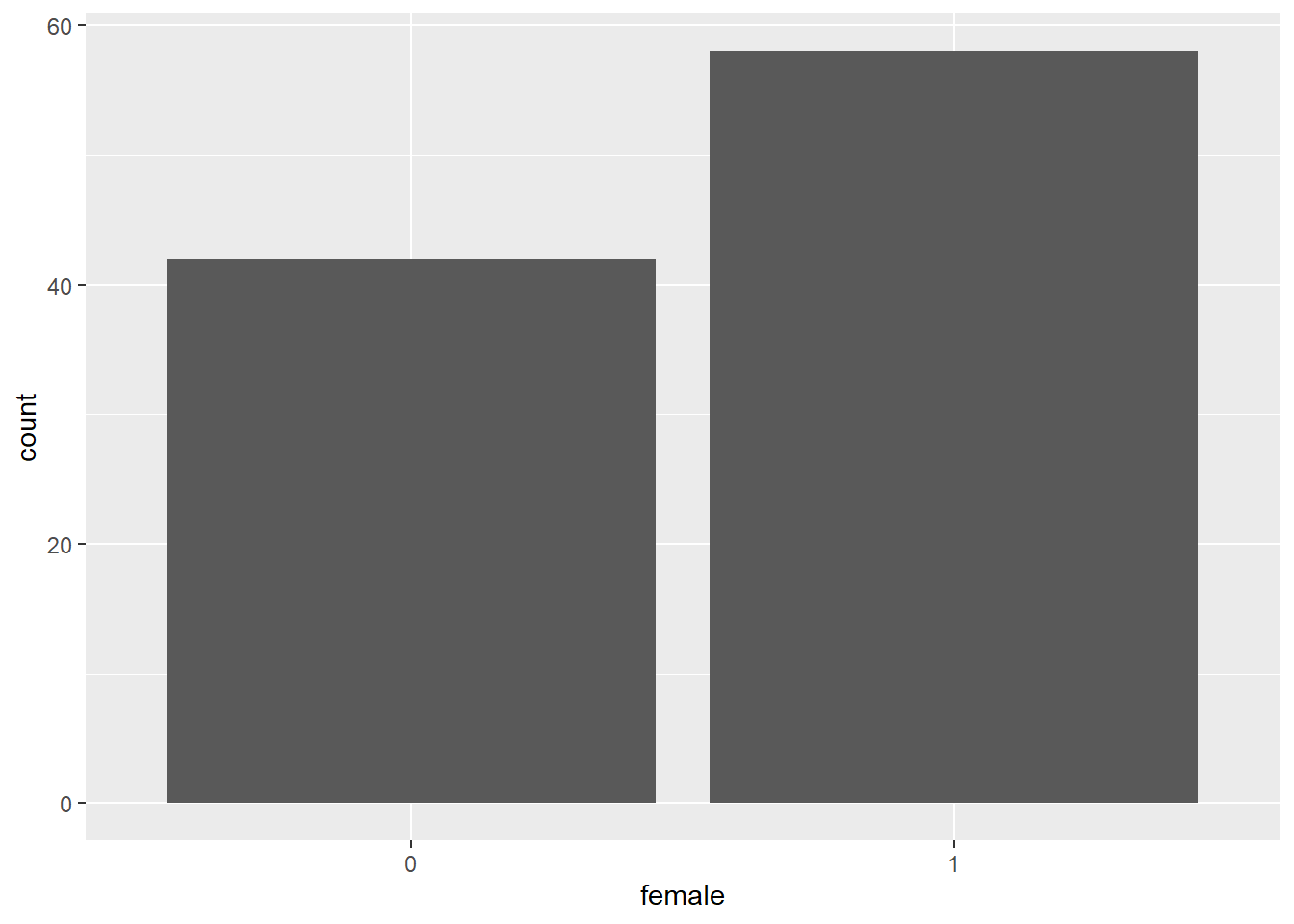< Two variables >

• geom_boxplot() for discrete X, continuous Y
``````ggplot(data = hsb, mapping = aes(female, mathach)) +
geom_boxplot() ``````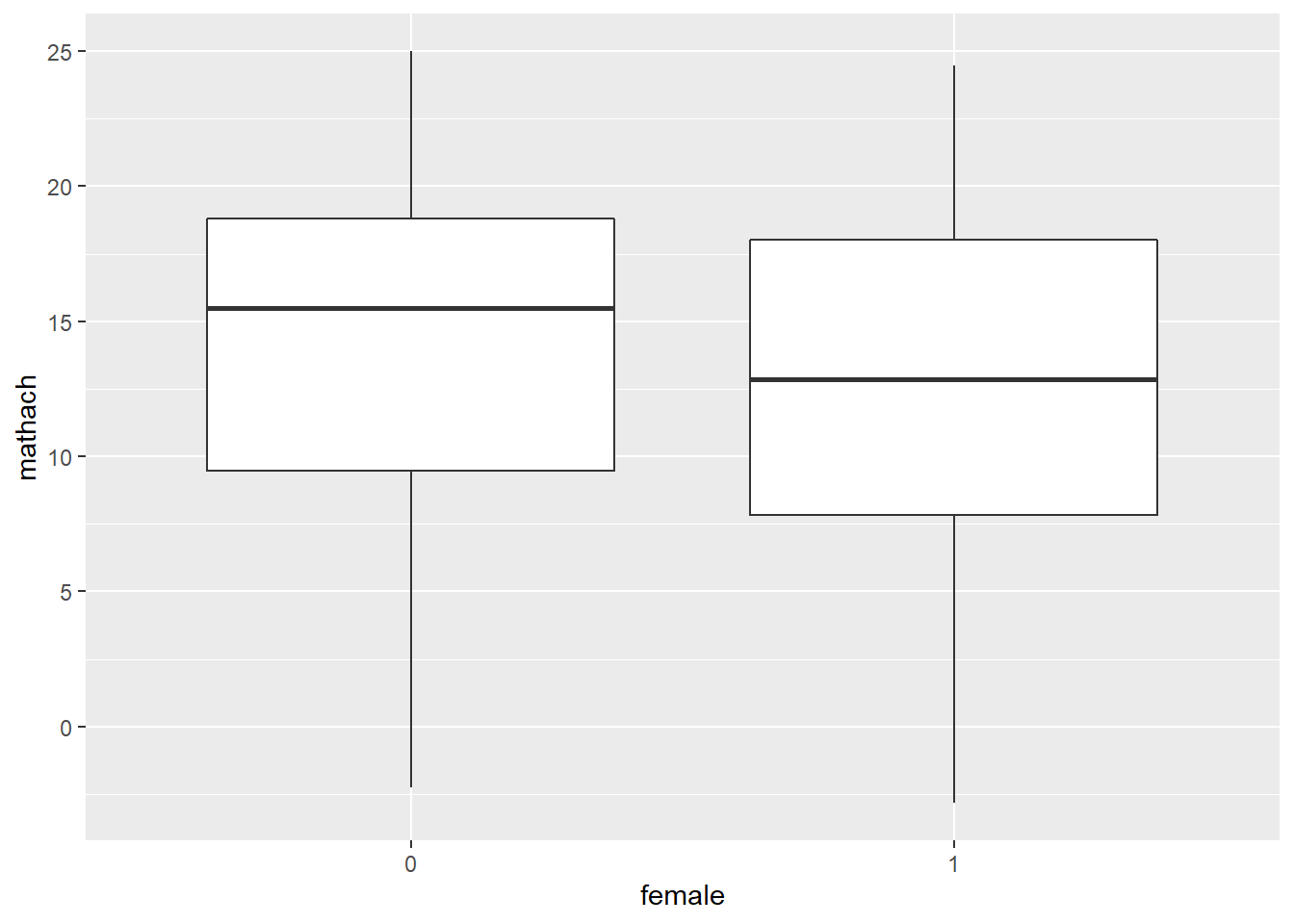• geom_point() for continuous X, Y
``````ggplot(data = hsb, mapping = aes(ses, mathach)) +
geom_point() ``````• geom_text() for continuous X, Y
``````## need 'label' aesthetic
ggplot(data = hsb, mapping = aes(ses, mathach)) +
geom_text(mapping = aes(label=id)) # plot labels ``````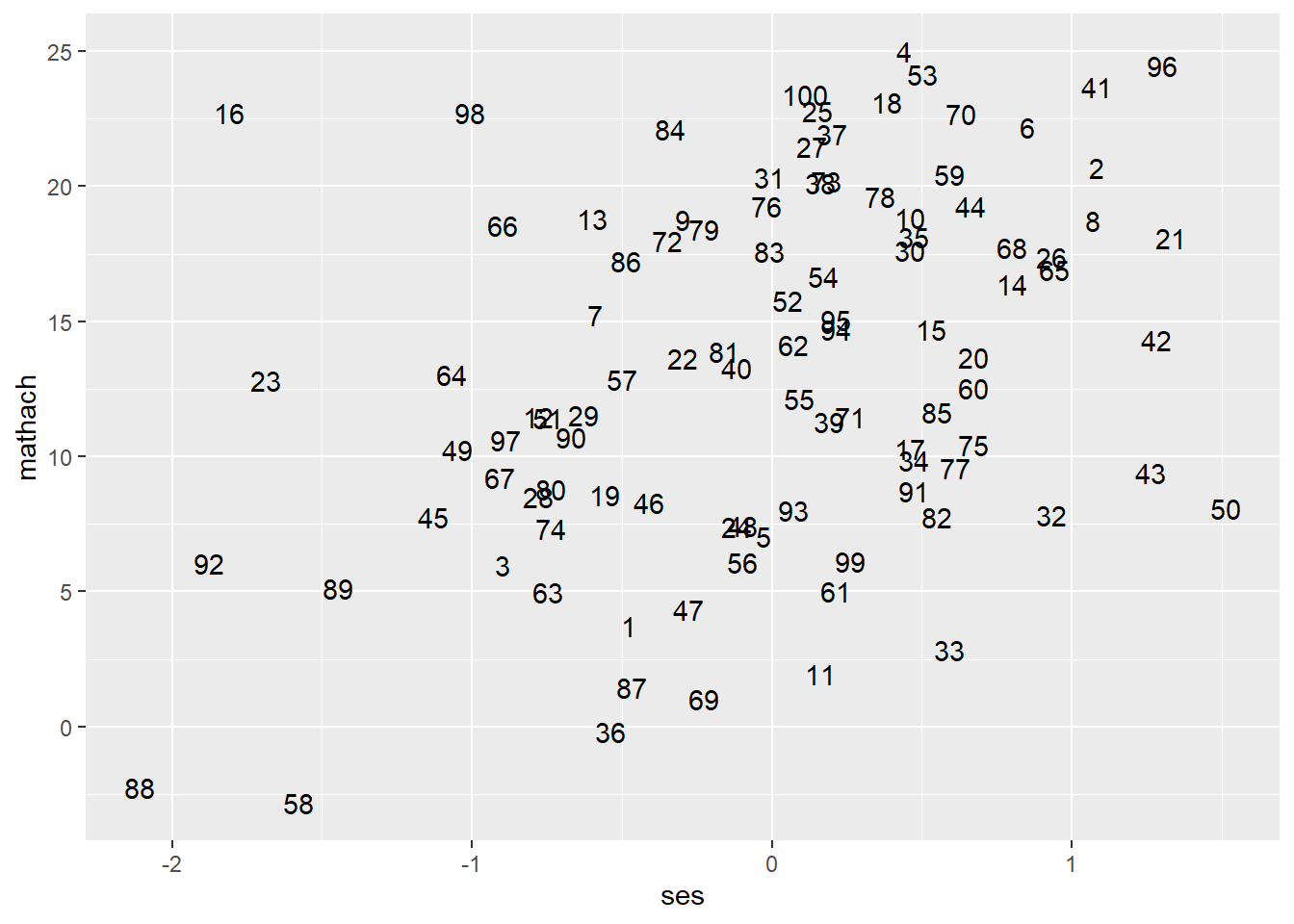``````## Add labels to a scatterplot
ggplot(data = hsb, mapping = aes(ses, mathach)) +
geom_point() +  # draw a scatterplot
geom_text(mapping = aes(label=id), nudge_x = 0.08) # add 'id' labels to points``````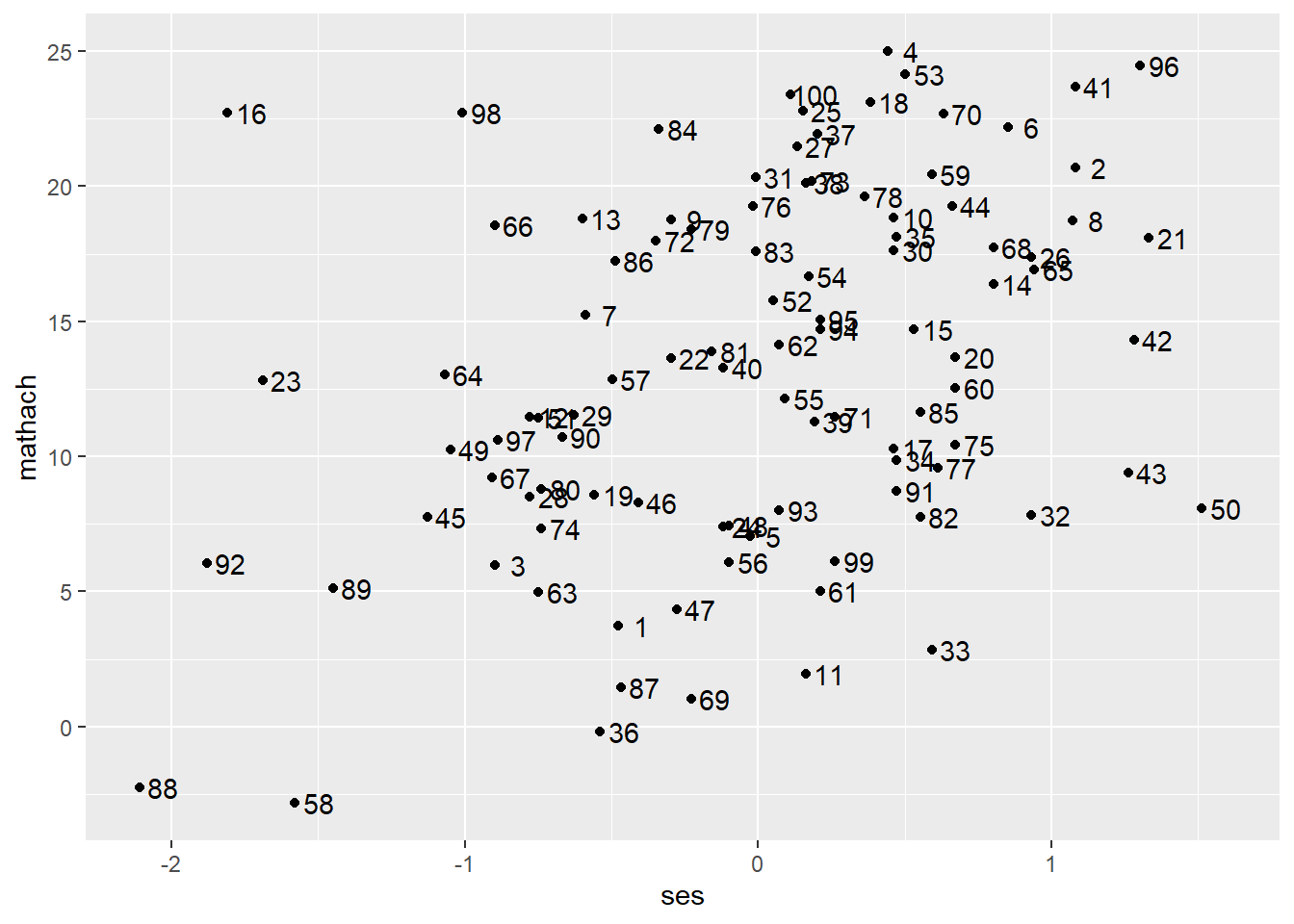• geom_smooth() for continuous X, Y
``````ggplot(data = hsb, mapping = aes(ses, mathach)) +
geom_point() +  # scatterplot
geom_smooth() # add a smooth line fitted to the data``````
``## `geom_smooth()` using method = 'loess' and formula 'y ~ x'``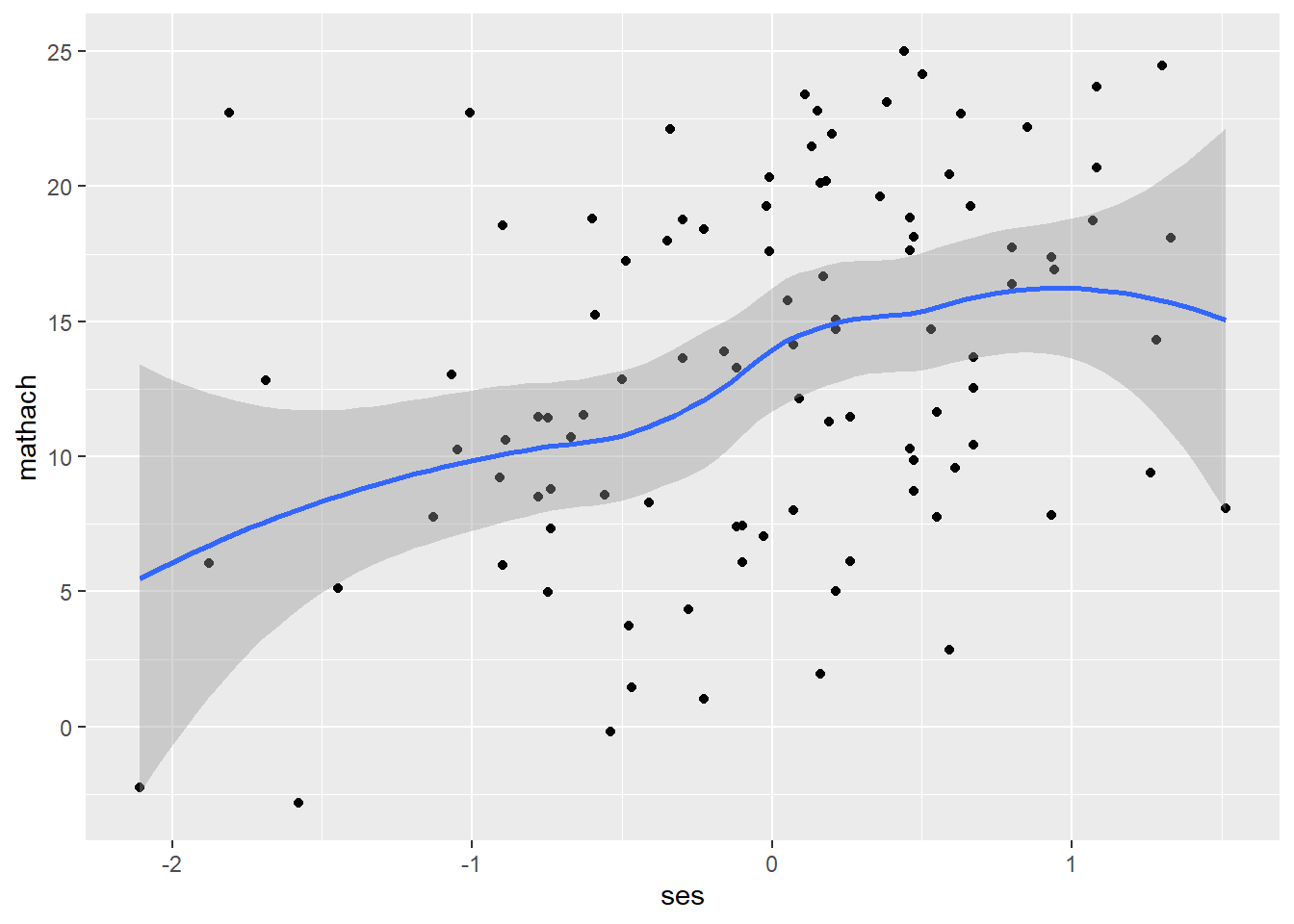< Line segments >

• geom_abline()
``````ggplot(data = hsb, mapping = aes(ses, mathach)) +
geom_point() +  # scatterplot
geom_abline(aes(intercept = 13.349, slope = 3.224)) # add a regression line``````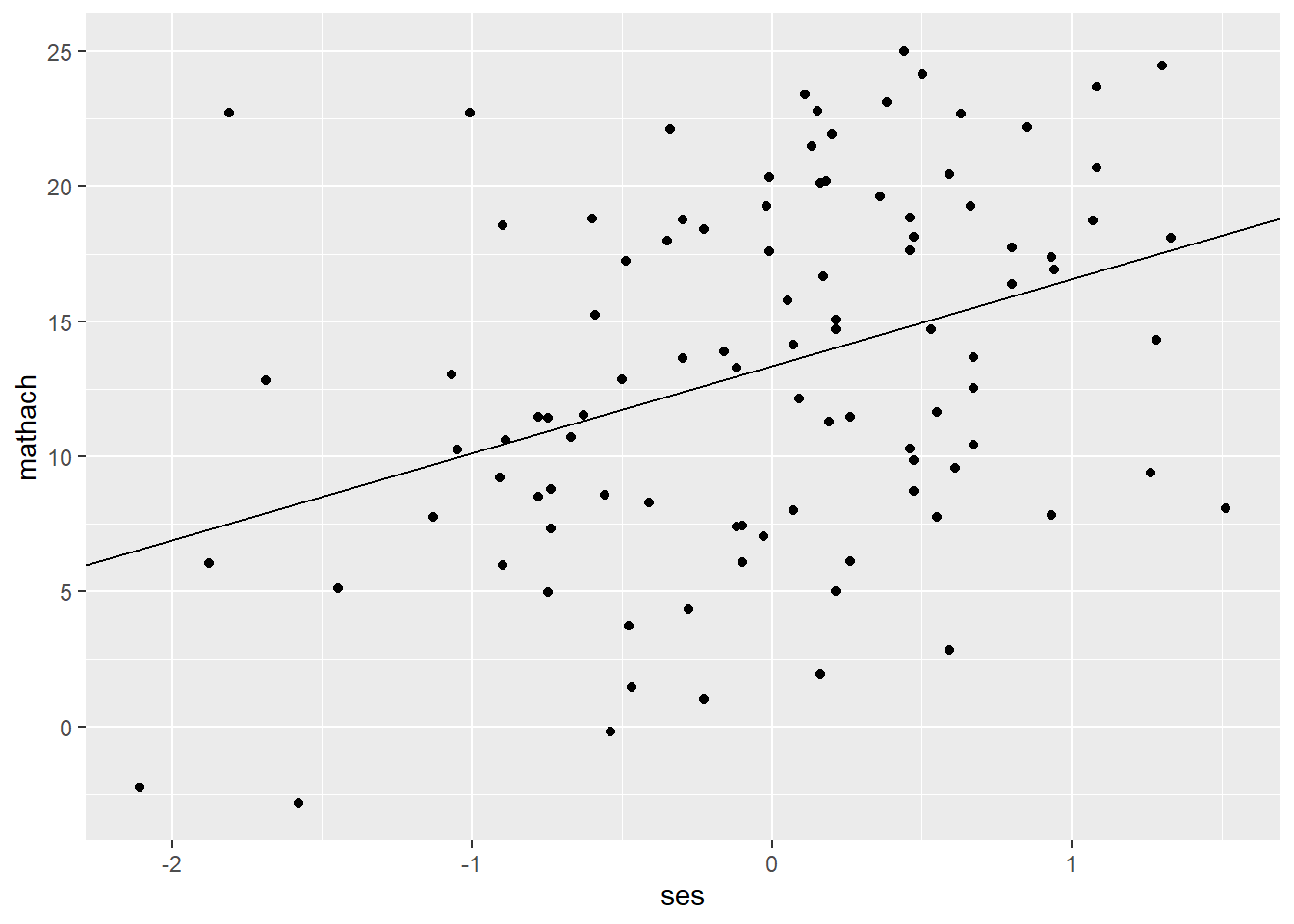``````## To add the regression equation
ggplot(data = hsb, mapping = aes(ses, mathach)) +
geom_point() +  # scatterplot
geom_abline(aes(intercept = 13.349, slope = 3.224)) + # add a regression line
geom_text(aes(label = "Mathach = 13.35 + 3.22 * SES + e"), x = 0.93, y = 13.5) # add the equation``````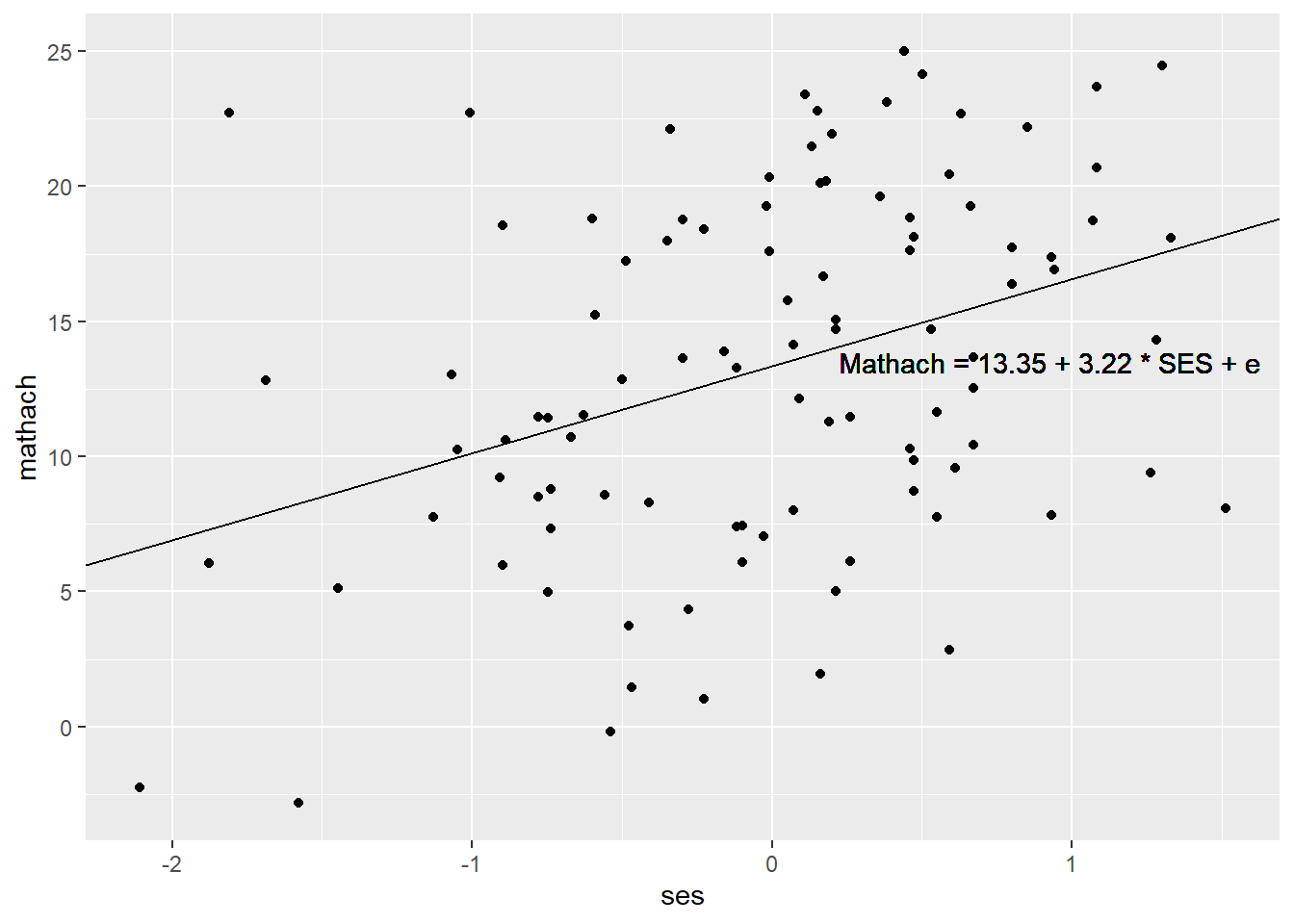## Color/Size/Shape/Linetype

• Color/Opacity

To map colors/opacity to a specific variable, add color/fill or alpha (which controls transparency) inside aes(). If you want to set colors/opacity, add them outside aes().

``````## Map colors/opacity to a variable
ggplot(data = hsb, mapping = aes(ses, mathach)) +   # create a ggplot object
geom_point(aes(color = female))   # map colors to 'female' (discrete)``````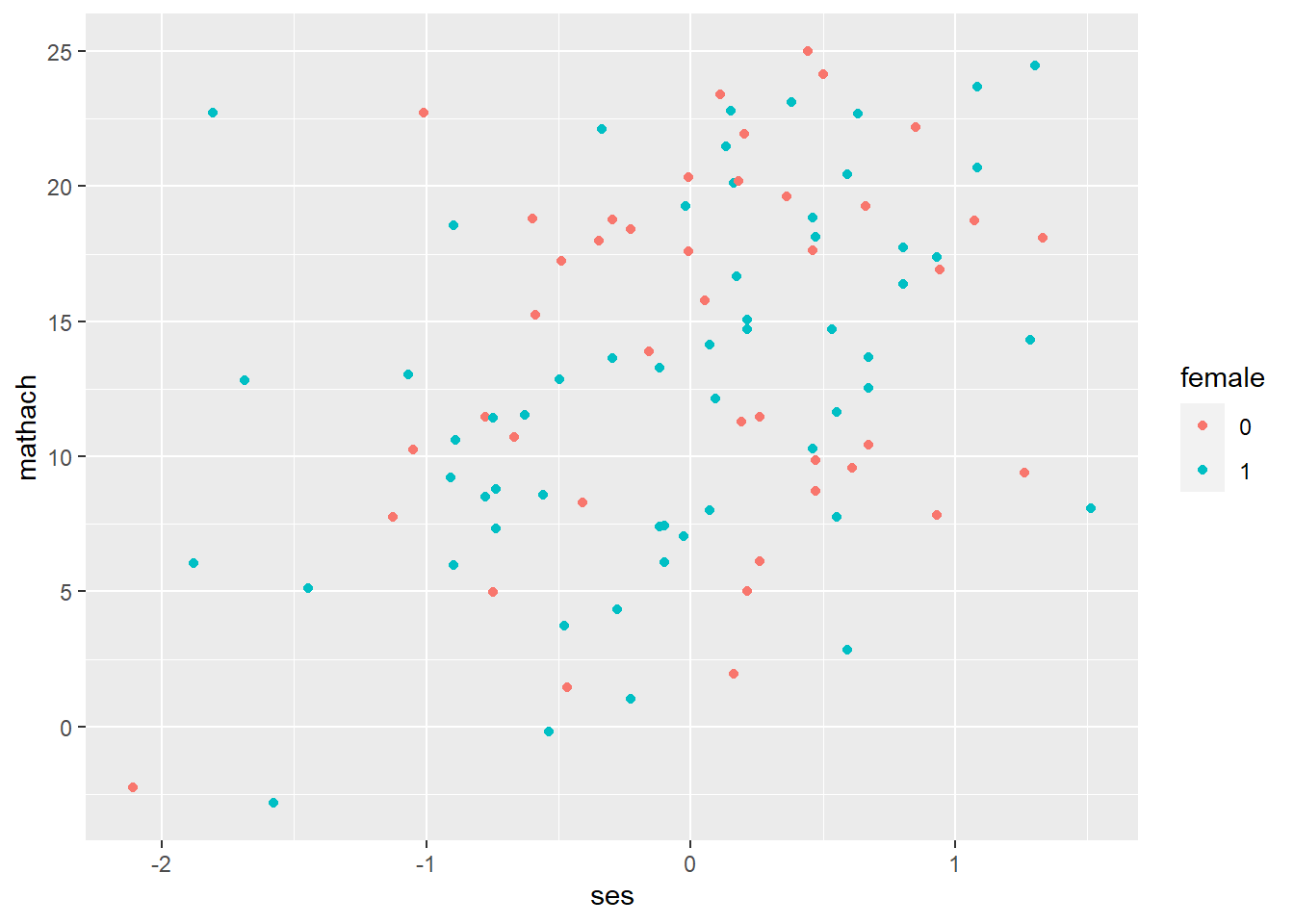``````ggplot(data = hsb, mapping = aes(ses, mathach)) +   # create a ggplot object
geom_point(aes(color = ses))   # map colors to 'ses' (continuous)``````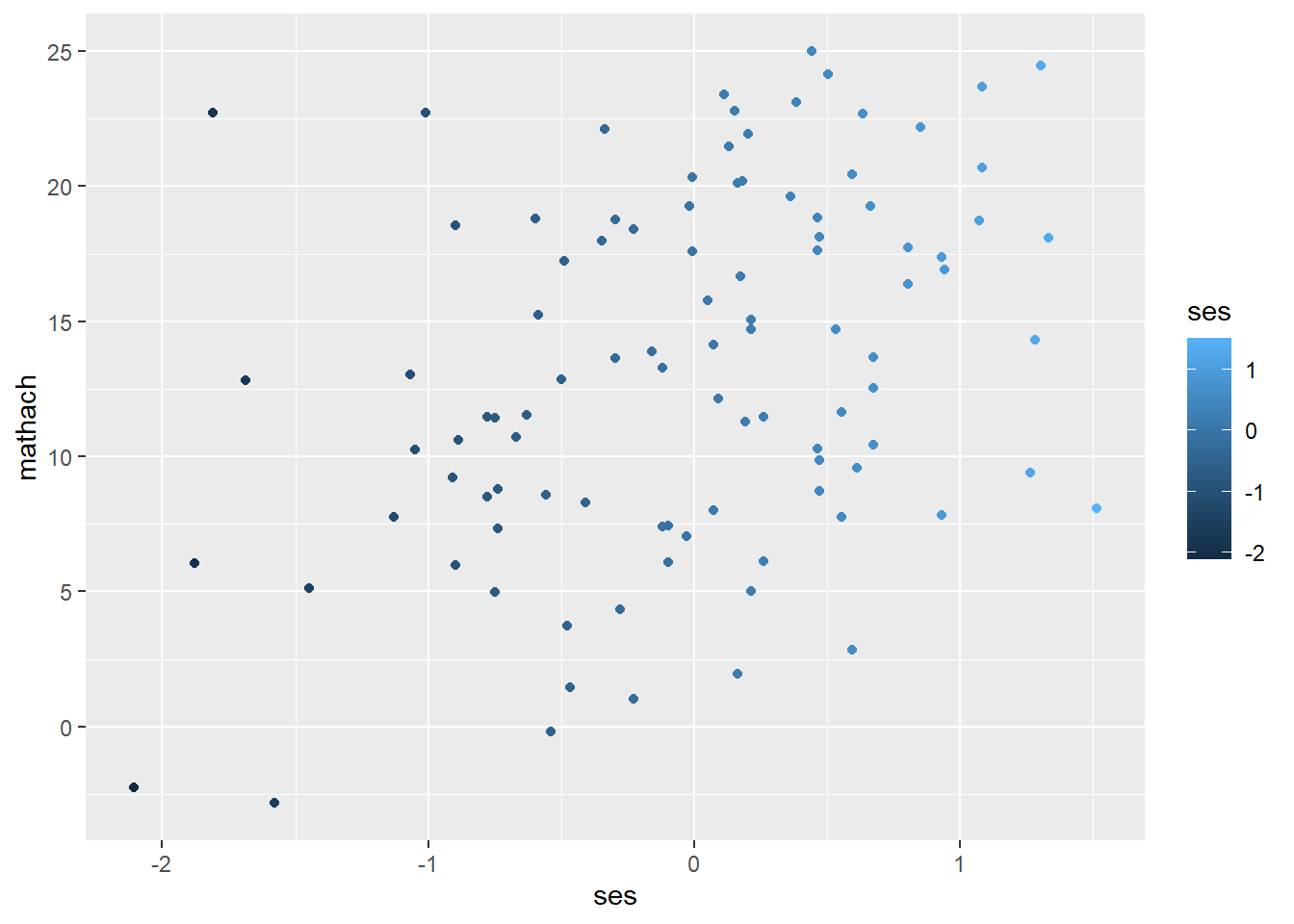``````ggplot(data = hsb, mapping = aes(ses, mathach)) +
geom_point(aes(alpha = ses))  # map opacify to 'ses (continuous)``````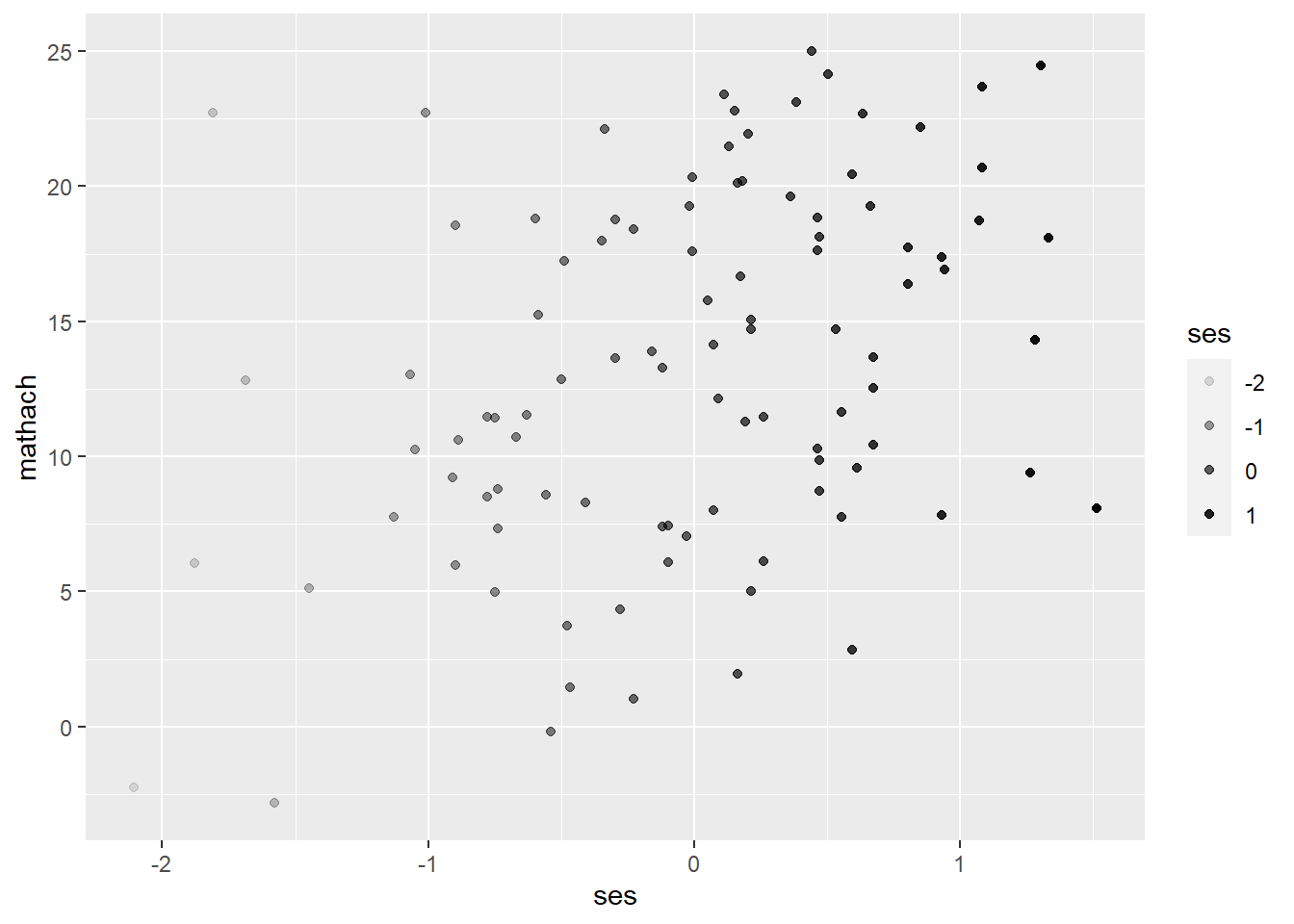``````ggplot(data = hsb, mapping = aes(mathach)) +
geom_density(aes(fill=female))  # map colors to 'female'``````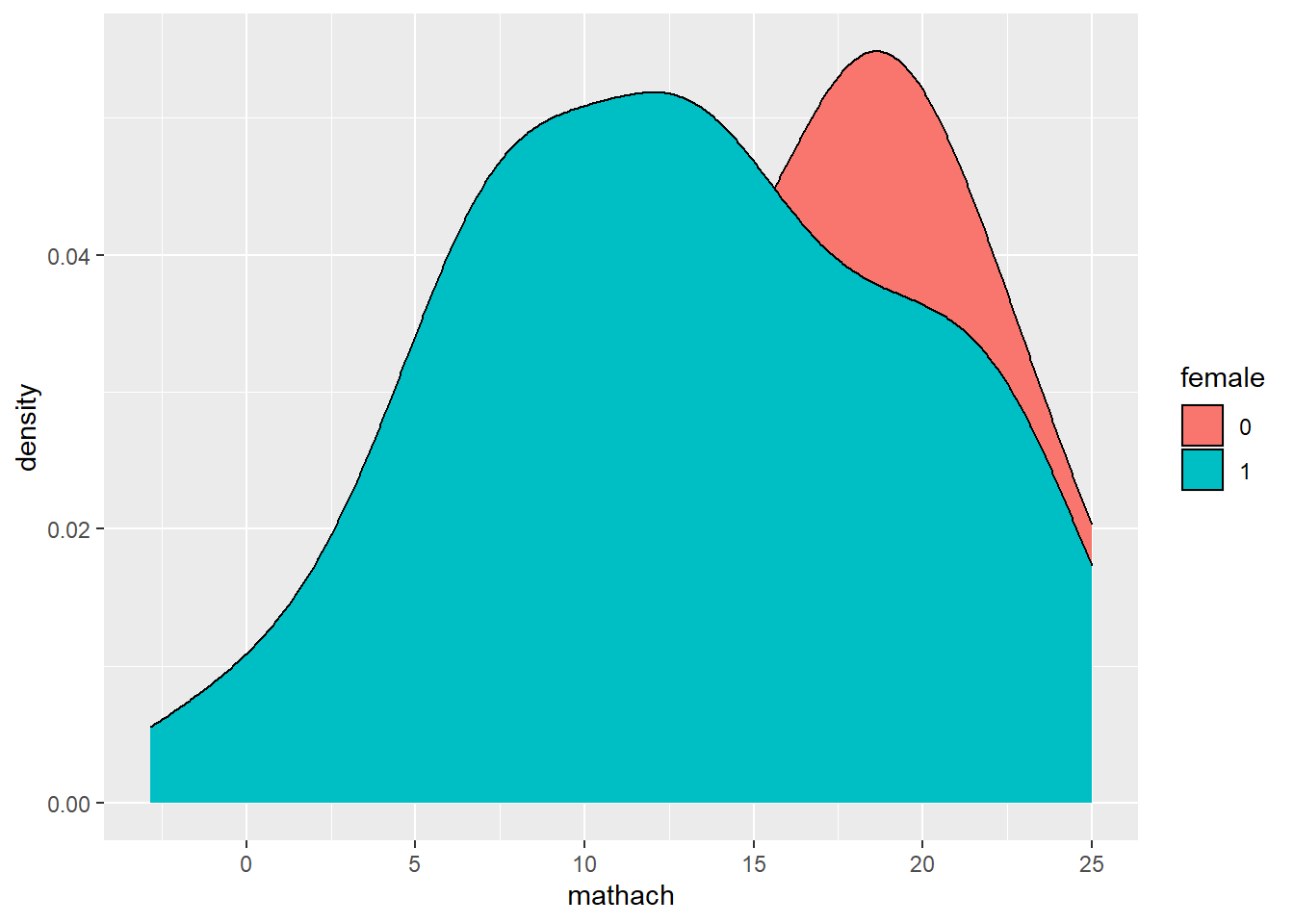``````ggplot(data = hsb, mapping = aes(mathach)) +
geom_density(aes(fill=female), alpha = 0.3) # map colors to 'female' & set alpha to 0.3``````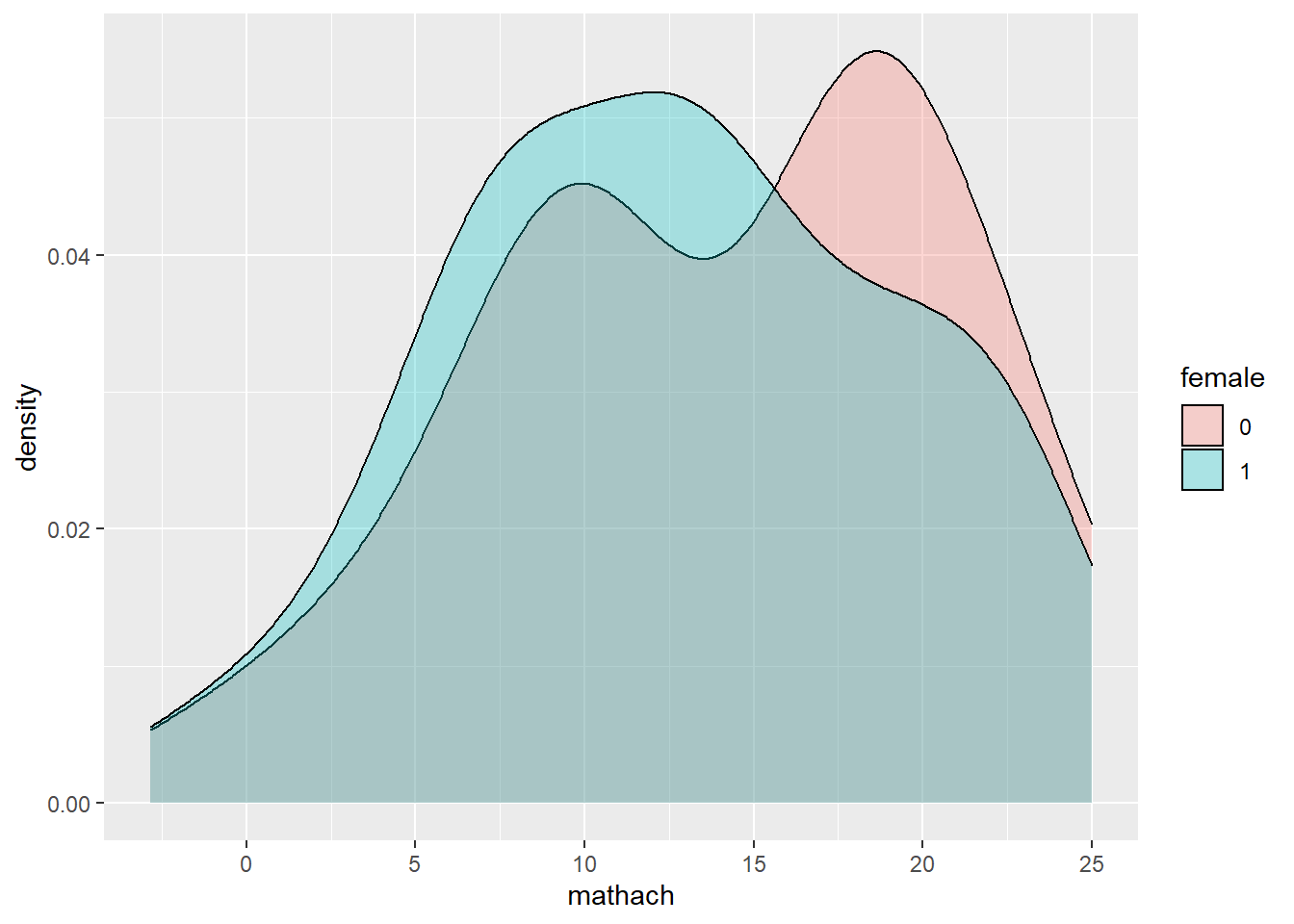``````## Set colors to a variable
ggplot(data = hsb, mapping = aes(ses, mathach)) +
geom_point(color = "orange")  # set color of points to orange``````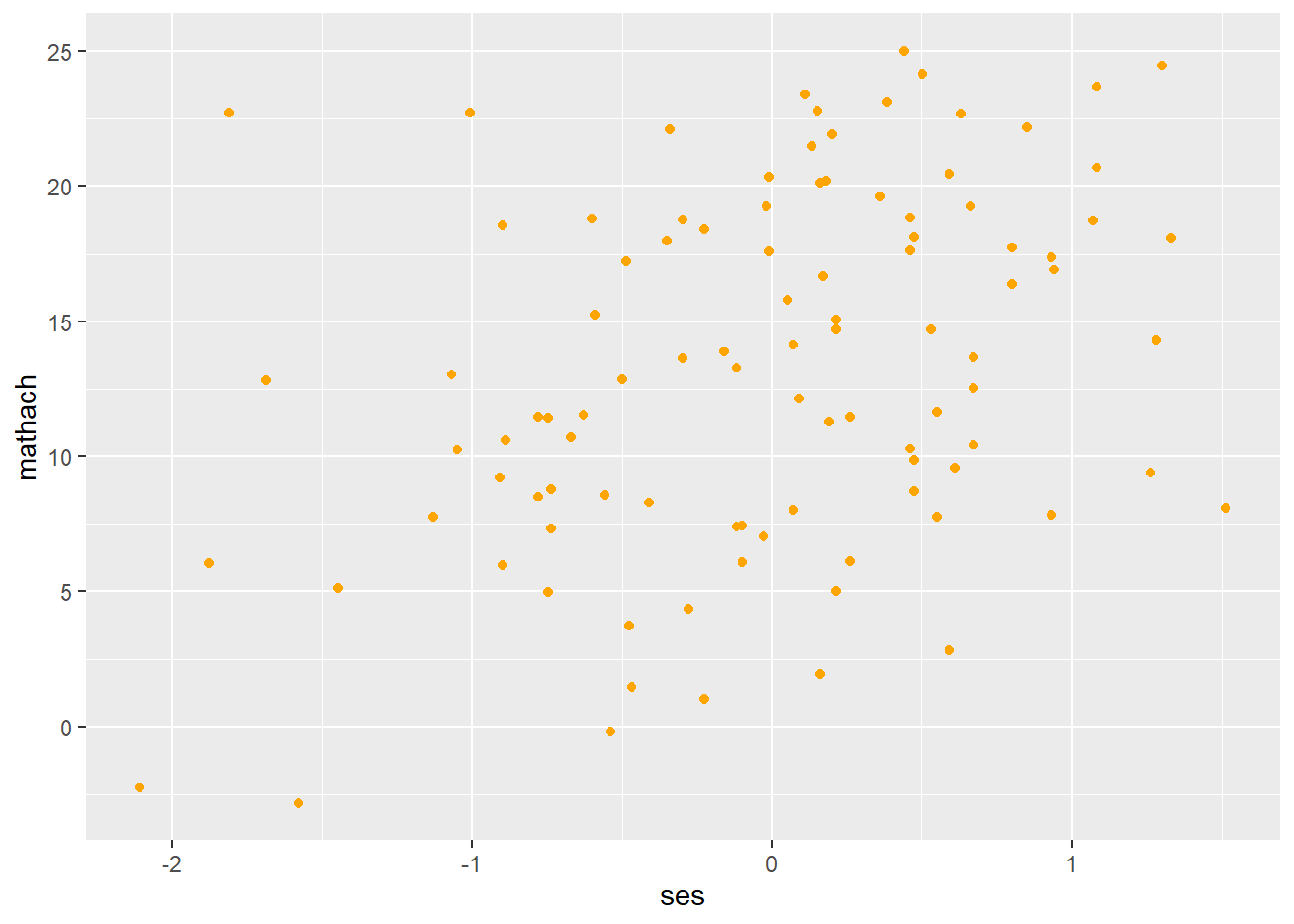``````ggplot(data = hsb, mapping = aes(mathach)) +
geom_density(fill = "pink")  # fill density area with pink``````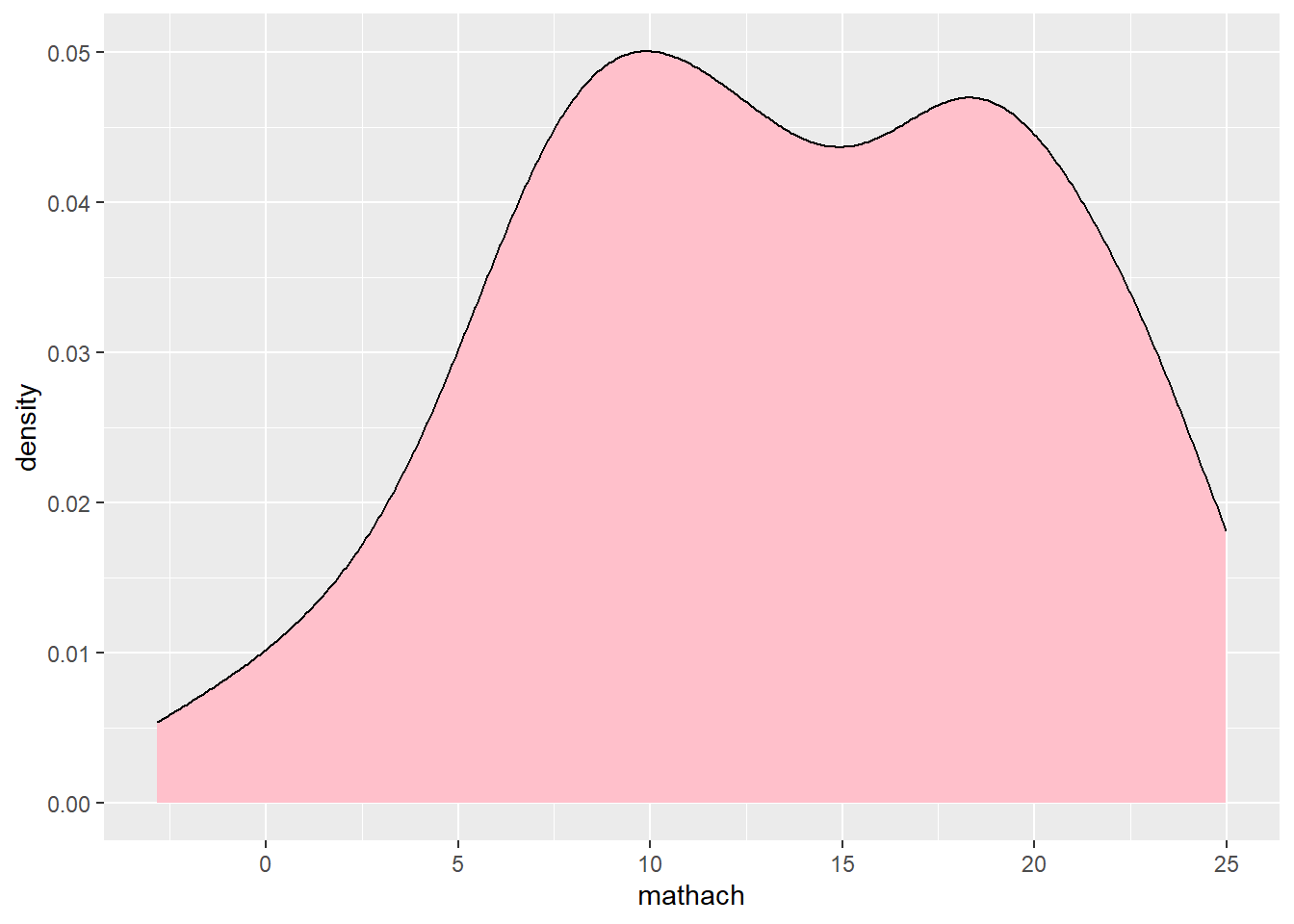• Size/Shape/Linetype

Likewise, we can set or map a variable to size/type of points/lines using size, shape, linetype arguments. Below are some examples of mapping these aesthetics to a variable.

``````## Map size/shape/linetype to a variable
ggplot(data = hsb, mapping = aes(ses, mathach)) +
geom_point(aes(size = ses)) # map size of points to 'ses'``````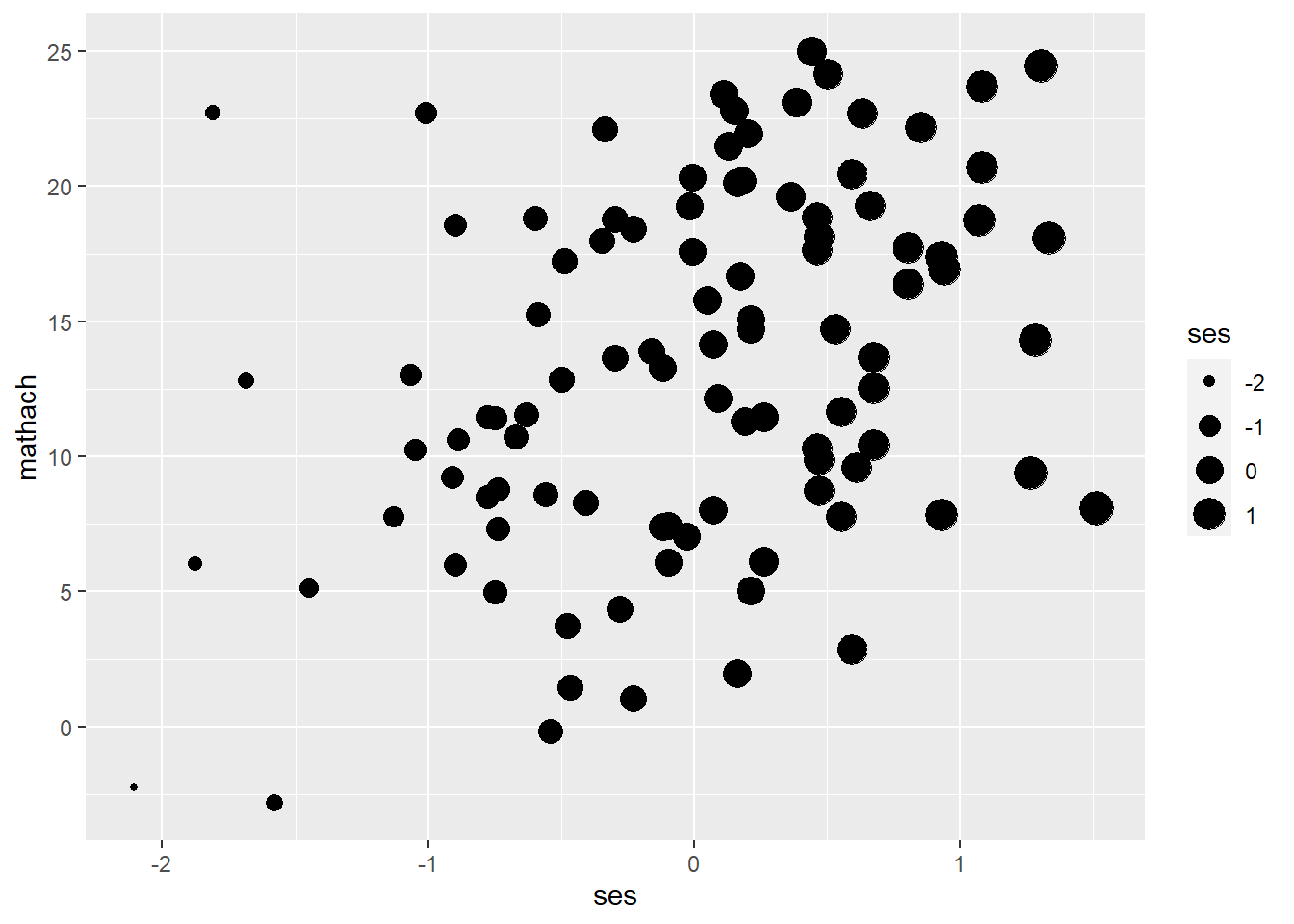``````ggplot(data = hsb, mapping = aes(ses, mathach)) +
geom_point(aes(shape = female)) # map shape of points to 'female'``````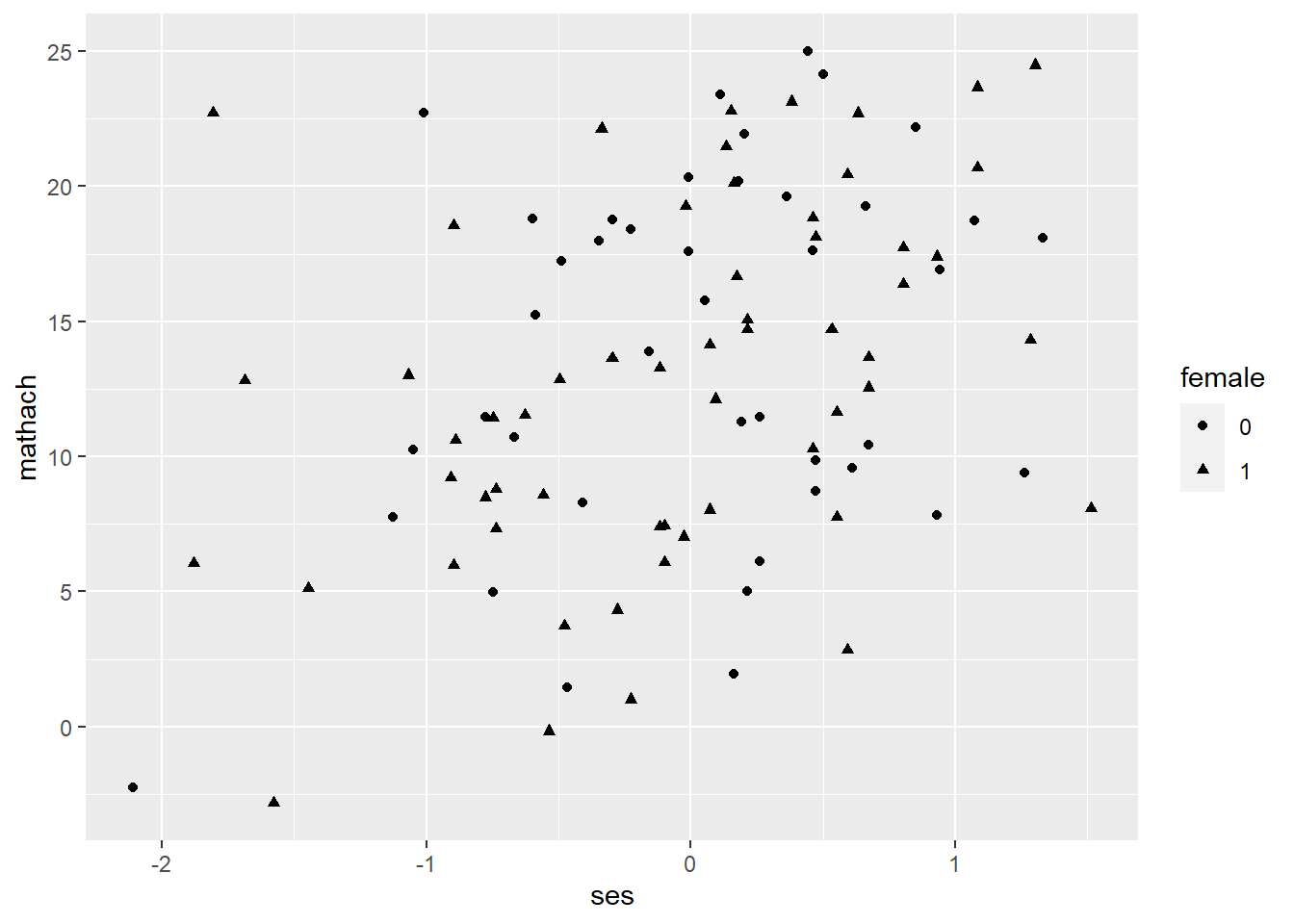``````ggplot(data = hsb, mapping = aes(ses, mathach)) +
geom_smooth(aes(linetype = female)) # map type of lines to 'female'``````
``## `geom_smooth()` using method = 'loess' and formula 'y ~ x'``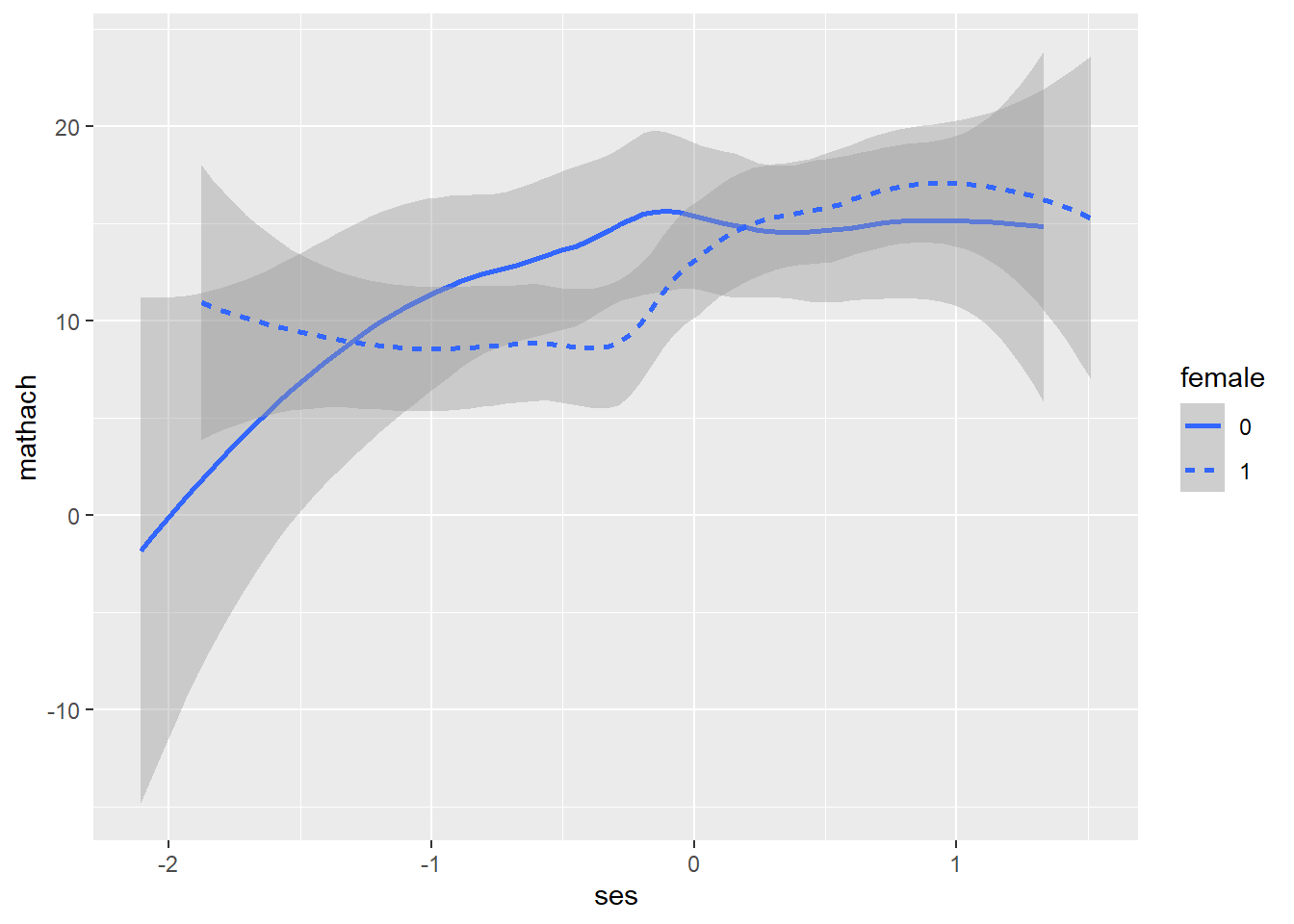Again, we need to include the arguments outside aes() to set the size and type of points/lines. To identify the type of points/lines, use the numbers/names shown in the figure below.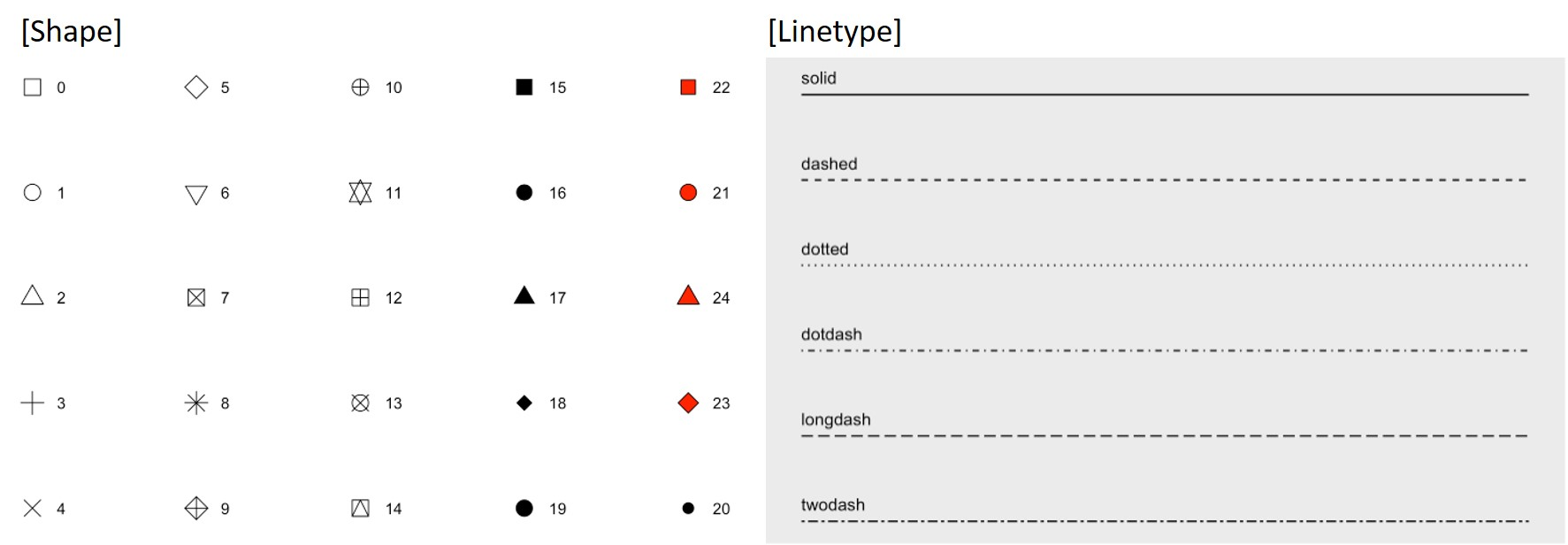``````## set size/type of points/lines
ggplot(data = hsb, mapping = aes(ses, mathach)) +
geom_point(size = 3) # increase the point size``````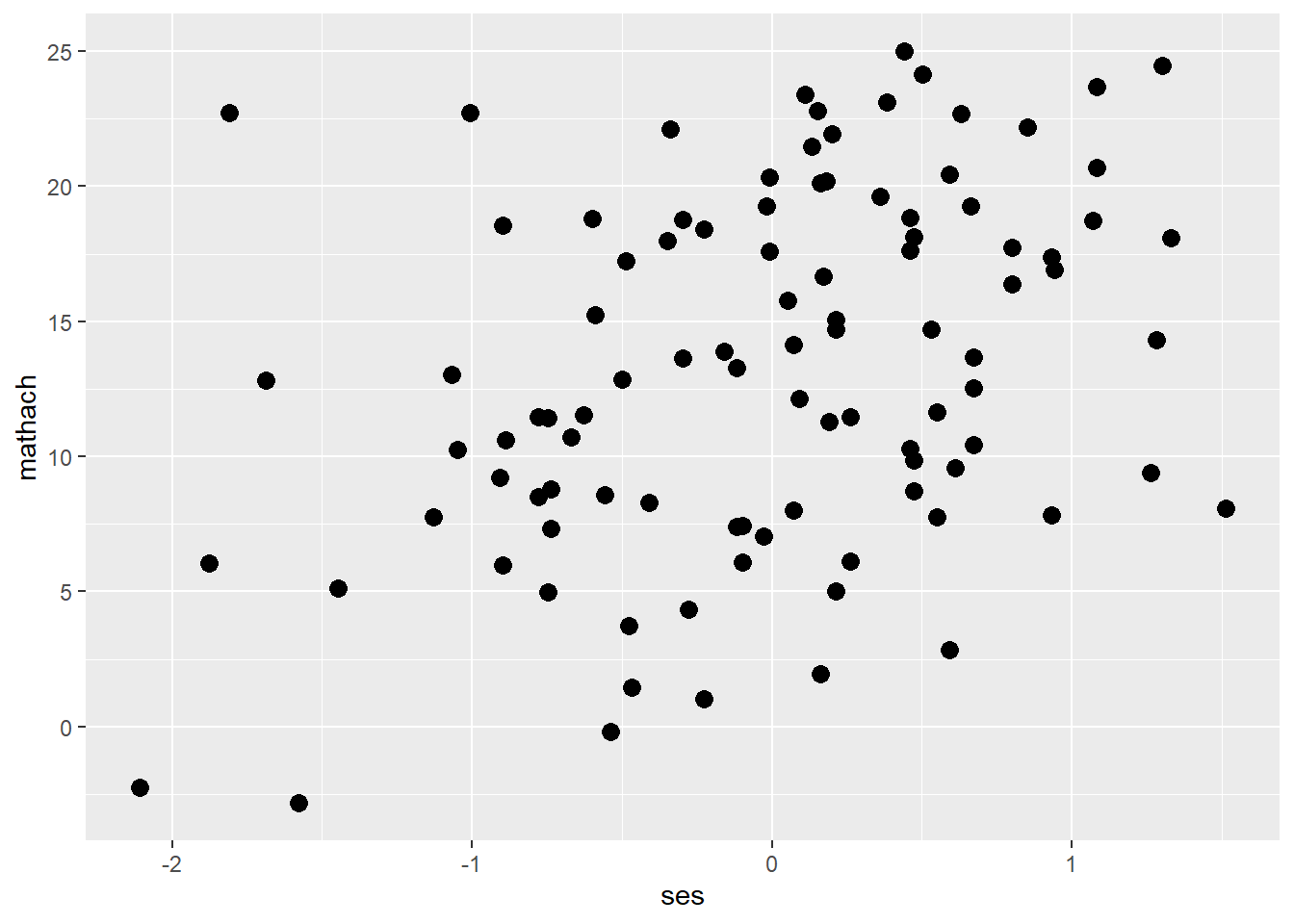``````ggplot(data = hsb, mapping = aes(ses, mathach)) +
geom_point(shape = 5) # change the shape of points``````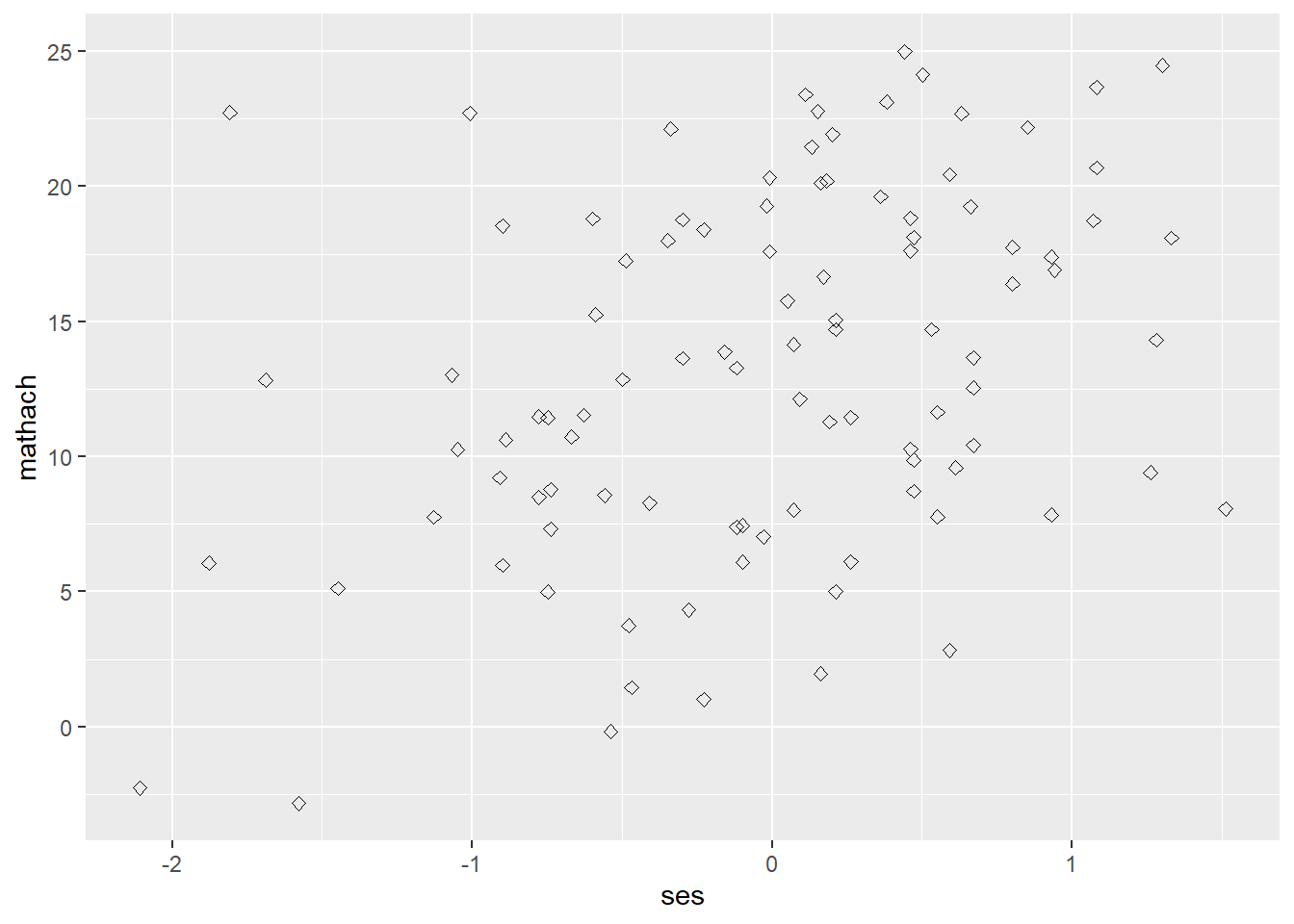``````ggplot(data = hsb, mapping = aes(ses, mathach)) +
geom_smooth(size = 3) # increase the line width``````
``## `geom_smooth()` using method = 'loess' and formula 'y ~ x'``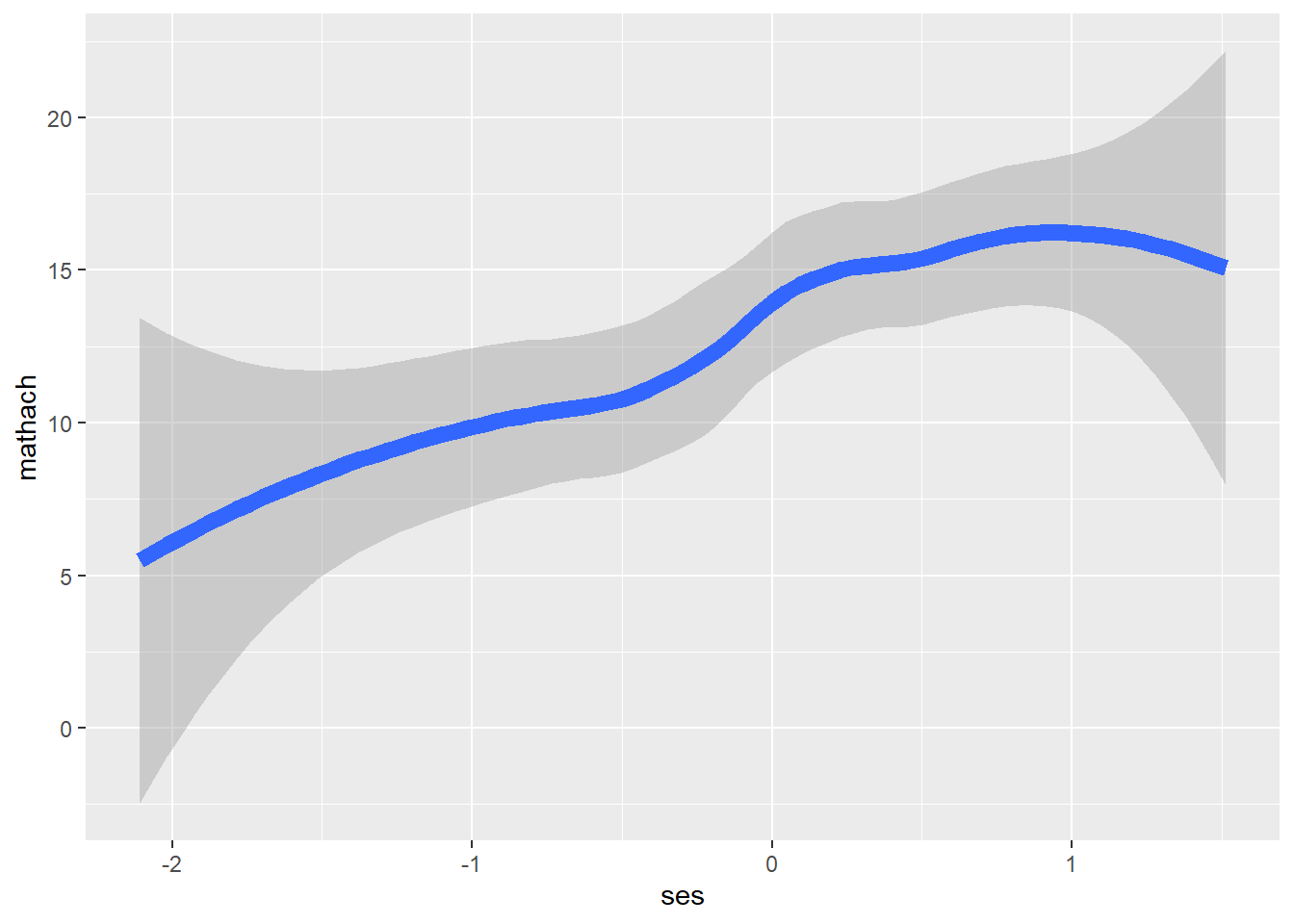``````ggplot(data = hsb, mapping = aes(ses, mathach)) +
geom_smooth(linetype = "longdash") # change the line type``````
``## `geom_smooth()` using method = 'loess' and formula 'y ~ x'``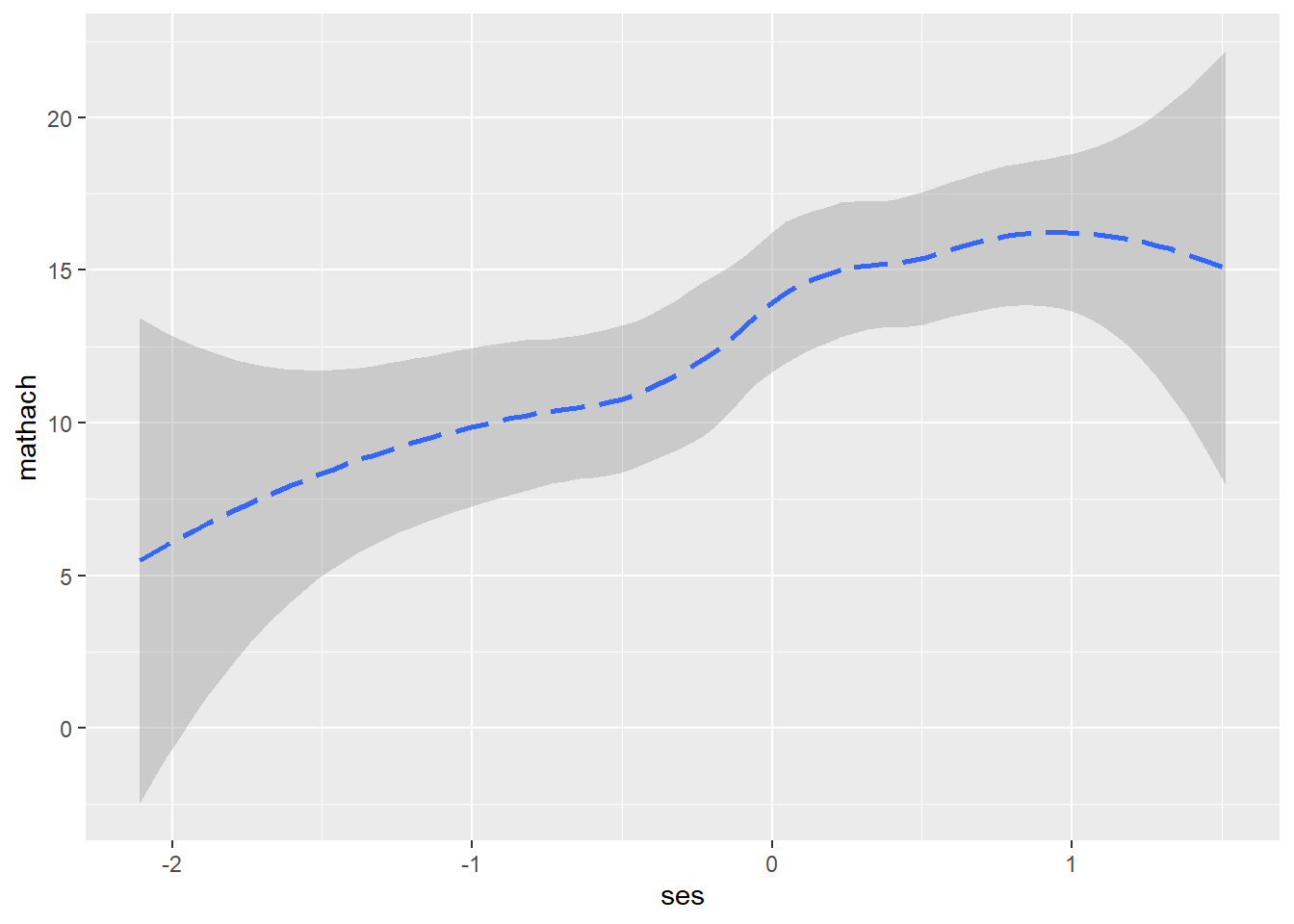## Facet

ggplot2::facet_wrap() and ggplot2::facet_grid() divide a plot into subplots based on the values of one or more discrete variables. facet_grid() is useful when you have two faceting variables. To facet the plot by a single variable with many levels, it is better to use facet_wrap().

``````## facet_wrap(vars(var_name))

ggplot(data=hsb, mapping = aes(ses, mathach)) +
geom_point() +
facet_wrap(vars(female), labeller = "label_both") ``````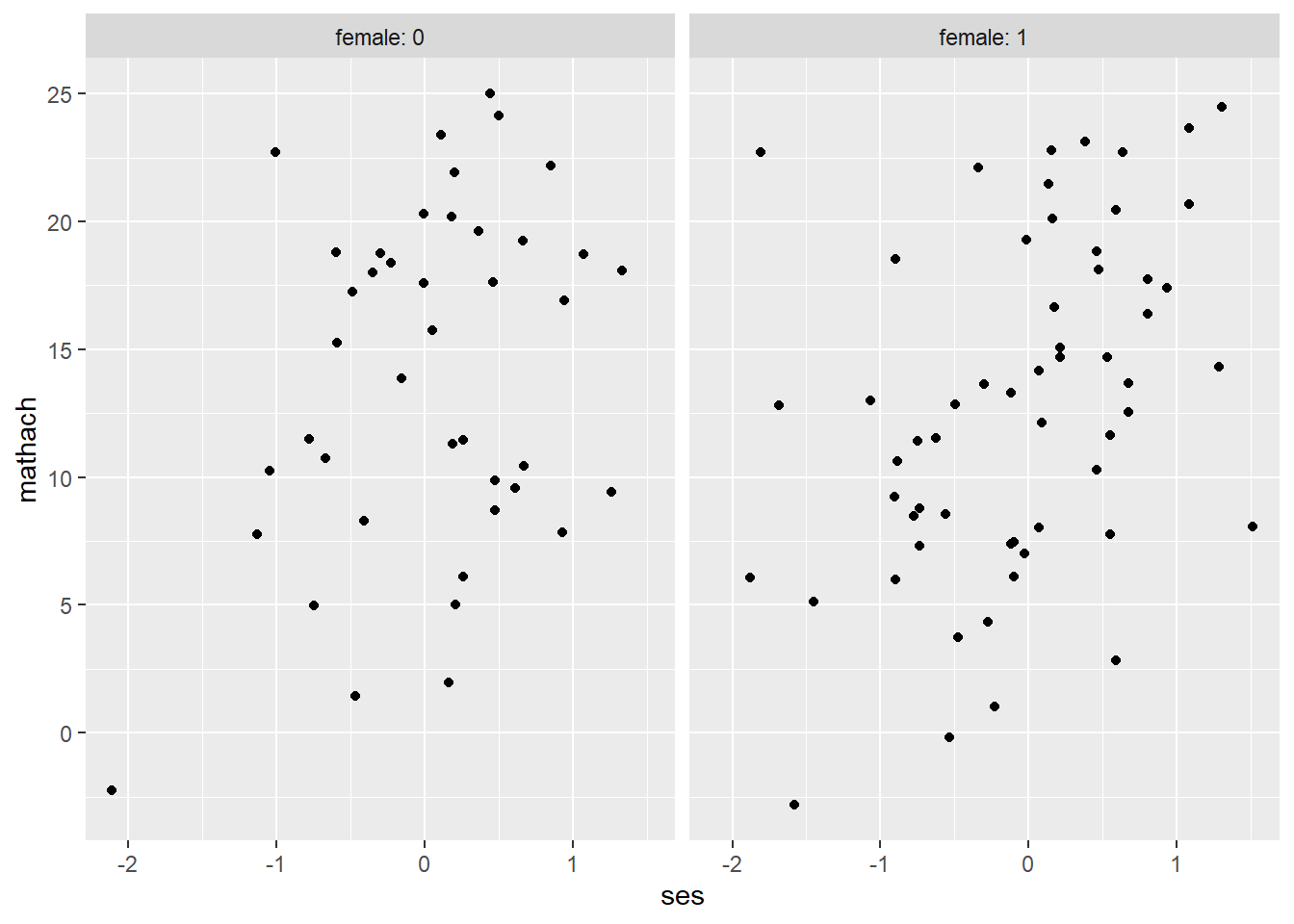``````ggplot(data=hsb, mapping = aes(ses, mathach)) +
geom_point() +
facet_wrap(vars(female), labeller = "label_both", nrow = 2)``````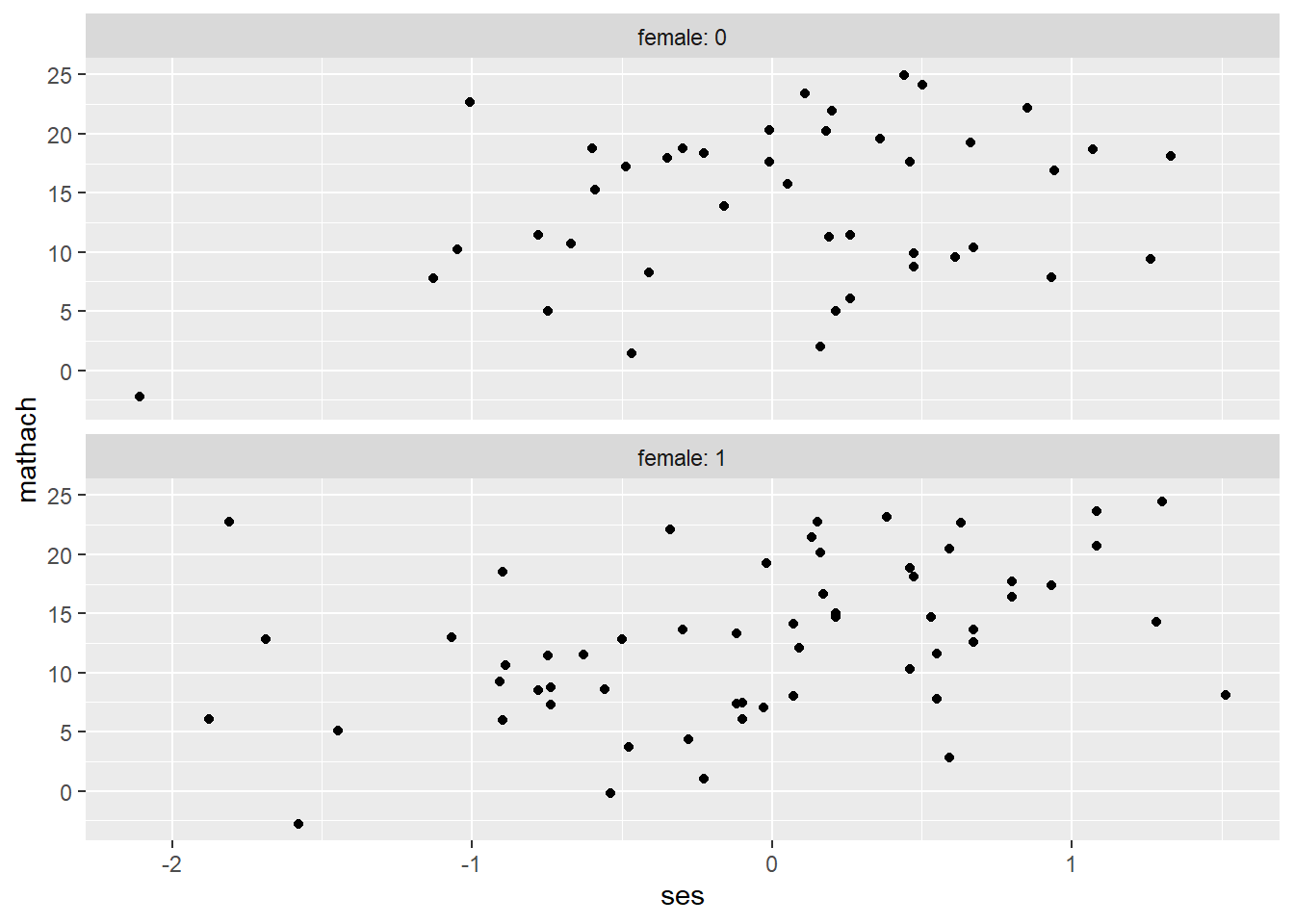``````## facet_grid(rows = vars(var1_name), cols = vars(var2_name))

ggplot(data=hsb, mapping = aes(ses, mathach)) +
geom_point() +
facet_grid(cols = vars(female), labeller = "label_both") ````````````ggplot(data=hsb, mapping = aes(ses, mathach)) +
geom_point() +
facet_grid(rows = vars(minority), cols = vars(female), labeller = "label_both")``````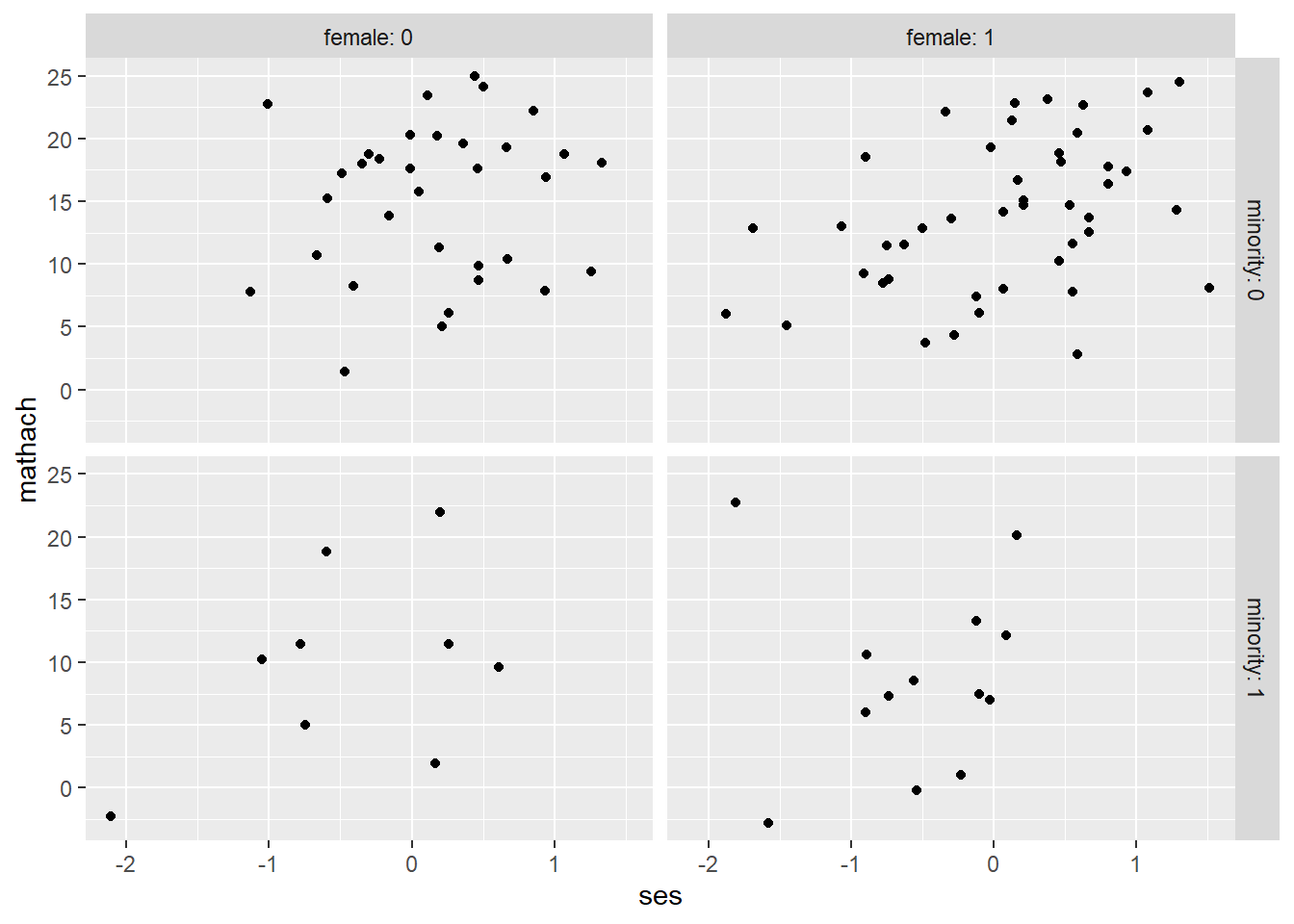We can also use a formula instead of vars() arguments as below.

``````ggplot(data=hsb, mapping = aes(ses, mathach)) +
geom_point() +
facet_grid(minority~female, labeller = "label_both")

ggplot(data=hsb, mapping = aes(ses, mathach)) +
geom_point() +
facet_grid(.~female, labeller = "label_both")

ggplot(data=hsb, mapping = aes(ses, mathach)) +
geom_point() +
facet_wrap(~female, labeller = "label_both") ``````

We can edit/add axes labels and titles using xlab()/ylab()/ggtitle, or labs function.

``````ggplot(data = hsb, mapping = aes(ses, mathach)) +
geom_point() +
xlab("Socioeconomic Status (SES)") +  # add x-axis label
ylab("Math achievement") +           # add y-axis label
ggtitle("Scatterplot of Math Achievement versus SES") # add title``````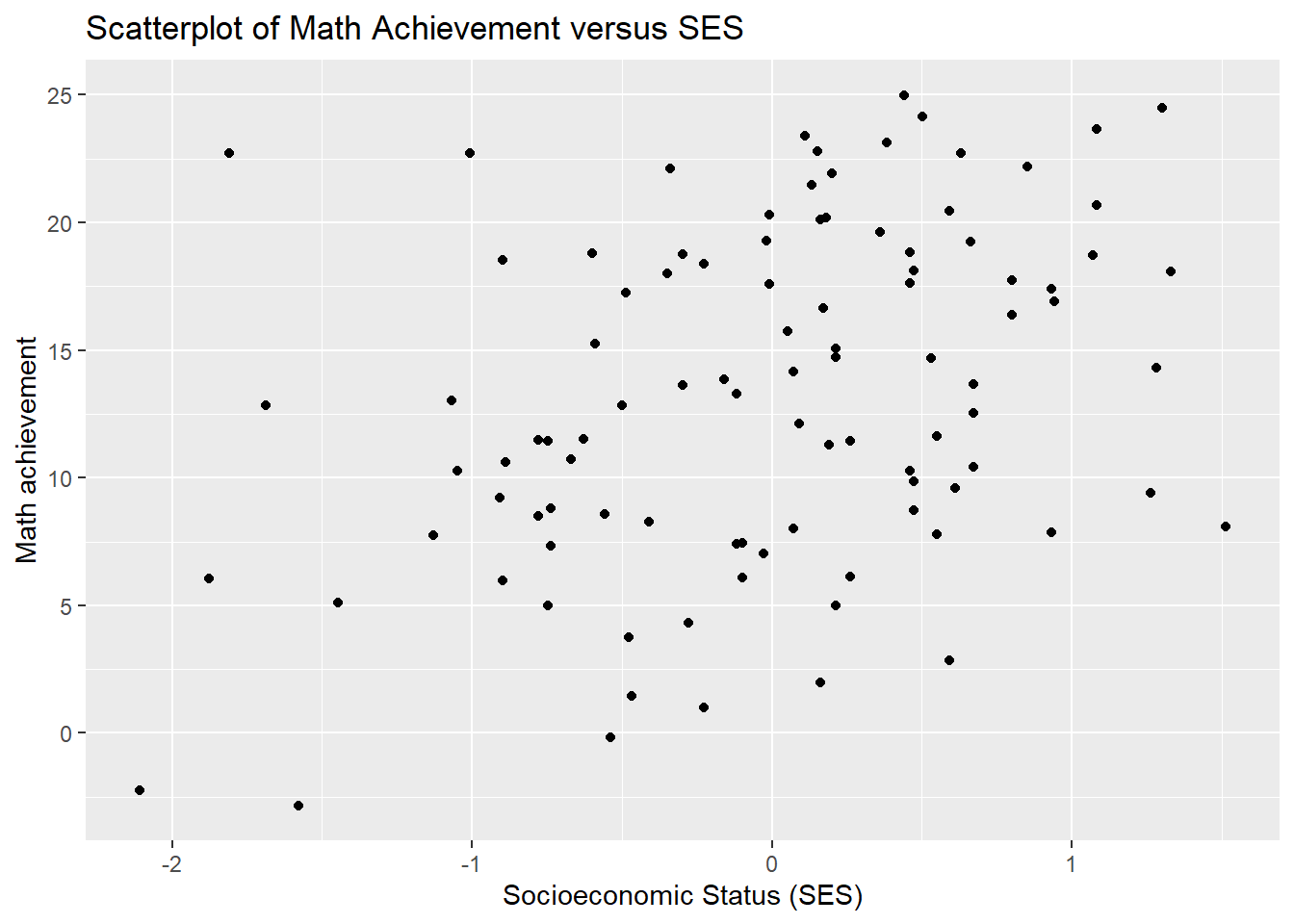``````# or

## labs(x="xlab", y="ylab", title="title")
ggplot(data = hsb, mapping = aes(ses, mathach)) +
geom_point() +
labs(x = "Socioeconomic Status (SES)",
y = "Math achievement",
title = "Scatterplot of Math Achievement versus SES")``````## Save Plot

We can conveniently save the resulting plot using ggsave(). This function saves the last plot produced.

``````ggsave("plotname.jpg")

## You can also modify plot size
ggsave("plotname.pdf", path = "folder_path", width = 4, height = 4)``````